# 第一章 古典密码学

## 基础定义

$\displaylines{一个密码体制满足以下条件的五元组(\mathcal{P},\mathcal{C},\mathcal{K},\varepsilon,\mathcal{D}) \\ \mathcal{P}表示明文空间 \\ \mathcal{C}表示密文空间 \\ \mathcal{K}表示密钥空间 \\ 加密规则e_\mathcal{K} \in \varepsilon \\ 解密规则d_\mathcal{K} \in \mathcal{D} \\ 并且加密、解密规则满足条件: 对每一个明文x\in \mathcal{P},均有d_\mathcal{\mathcal{K}}(e_\mathcal{K}(x))=x}$

## 移位密码

$\displaylines{令P=C=K=\mathbb{Z}_{26},对0\leq K \leq 25,任意x,y\in\mathbb{Z}_{26} \\ e_k(x) = (x+K)\mod26 \\ d_k(y) = (y - K)\mod26}$

## 代换密码

$\displaylines{令P=C=\mathbb{Z}_{26}。K是由26个数字0,1...,25的所有可能的置换组成。 \\ 对任意的置换\pi\in K，定义 \\ e_\pi(x)=\pi(x) \\ d_\pi(y)=\pi^{-1}(y) \\ 其中\pi^{-1}表示\pi函数的逆变换}$

## 密码分析初步

### Kerchhoff原则

Kerchhoff原则：密码系统的安全性不依赖于加密体制或算法的保密，而依赖于密钥的安全性。

• 保护密钥比保护算法更加容易
• 更换密钥比更换算法要更加容易
• 加密算法需要统一，便于用户应用
• 加密算法公开有助于承受公开的钻研和分析，从而提高算法的防御力。同时使公开设计使标准的建立成为可能

### 典型攻击模型

• 唯密文攻击：敌手只拥有（某些个）密文串$$y$$
• 已知明文攻击：敌手拥有（某些个）明文串$$x$$及其对应的密文串$$y$$
• 选择明文攻击：敌手可以自由选择（某些个）明文串$$x$$并获得对应的密文串$$y$$
• 选择密文攻击：敌手可以自由选择（某些个）密文串$$y$$并获得对应的明文串$$x$$

## 仿射密码

$\displaylines{令P=C=\mathbb{Z}_{26},且 K = {(a,b)\in\mathbb{Z}_{26}\times\mathbb{Z}_{26}:gcd(a,26)=1} \\ 对任意的K=(a,b)\in K,{\,\,}x,y\in\mathbb{Z}_{26},定义 \\ e_K(x) = (ax+b)\mod26 \\ d_K(y) = a^{-1}(y-b)\mod26 \\ 其中a^{-1}表示a的逆元（aa^{-1}=1\mod26）}$

• 同余方程定理

$$a\in\mathbb{Z}$$，则同余方程$$a \cdot x \equiv b \mod m$$有唯一解$$x\in\mathbb{Z}$$的充分必要条件是$$gcd(a,m)=1$$

• 同余方程定理 >$$\mathbb{Z}_m$$中与$$m$$互素的数的个数成为$$m$$的欧拉函数，记为$$\phi(m)$$
• 基本算术定理与欧拉函数计算 >如果不计因子顺序，任意整数$$m$$的素因子分解是唯一的。 >设$$m = \Pi^n_{i=1}p^{e_i}_{i}$$这里$$p_i$$均为素数且互不相同，$$e_i>0,{\,\,}1\leq i \leq n$$，则$$\phi(m)=\Pi^n_{i=1}(p^{e_i}_i-p^{e_i}_{i-1})$$

## 维吉尼亚密码

$\displaylines{设m是一个正整数。定义P=C=K=(\mathbb{Z}_{26})^m。 \\ 对任意的密钥K=(k_1,k_2,...,k_m)，定义 \\ e_K(x_1,x_2,...,x_m)=(x_1+k_1,x_2+k_2,...,x_m+k_m) \\ d_K(y_1,y_2,...,y_m)=(y_1-k_1,y_2-k_2,...,y_m-k_m)}$

# -*- coding:utf-8 -*-

# 读取明文
t = []
with open("./plaintext.txt", "r", encoding="utf-8") as plaintext:
text = text.strip()
for chr in text:
t.append(chr)
return t

# 读取密钥
key = ""
with open("./key.txt", "r", encoding="utf-8") as f:
return key

# 写入密文
def writeCphertext(text):
with open("./cphertext.txt", "w", encoding="utf-8") as cphertext:
cphertext.write(text)

# 维吉尼亚密码加密过程
def vigenereEnc(plaintext, key):
cphertext = ""
key_length = len(key)
upper_key = key.upper()
index = 0 # 密钥下标
for iter in plaintext:
if iter.isalpha():
# 如果是大写字母
if iter.isupper():
diff = 'A'
else:
diff = 'a'
cphertext += chr(ord(diff) + ((ord(iter) - ord(diff)) + ord(upper_key[index % key_length]) - ord('A')) % 26)
index += 1
else:
cphertext += iter
return cphertext

# 维吉尼亚密码解密过程
def vigenereDec(cphertext, key):
plaintext = ""
key_length = len(key)
upper_key = key.upper()
index = 0 # 密钥下标
for iter in cphertext:
if iter.isalpha():
if iter.isupper():
diff = 'A'
else:
diff = 'a'
plaintext += chr(ord(diff) + ((ord(iter) - ord(diff)) - ord(upper_key[index % key_length]) - ord('A')) % 26)
index += 1
else:
plaintext += iter
return plaintext

if __name__ == "__main__":
cphertext = vigenereEnc(plaintext, key)
writeCphertext(cphertext)

• Kasiski测试法 原理：如果两个相同的明文段被加密成相同的密文段，则这两个密文段之间的间距以很大概率为密钥长度的整数倍。 方式：搜索长度至少为3的相同密文段，确定其离起始密文段的距离, 进而猜测长度 m 为这些距离的最大公因子的因子
• 重合指数法

$\displaylines{I_c(x)=\frac{\Sigma^{25}_{i=0} \begin{bmatrix} f_i \\ 2 \\ \end{bmatrix}} {\begin{bmatrix} n \\ 2 \\ \end{bmatrix} } = \frac {\Sigma^{25}_{i=0}f_i(f_i-1)} {n(n-1)}}$

# -*- coding:utf-8 -*-

import math
import Vigenere

# 字母频数表
dic_freq = {'A': 8.167, 'B': 1.492, 'C': 2.782, 'D': 4.253,
'E': 12.702, 'F': 2.228, 'G': 2.015, 'H': 6.094,
'I': 6.966, 'J': 0.153, 'K': 0.772, 'L': 4.025,
'M': 2.406, 'N': 6.749, 'O': 7.507, 'P': 1.929,
'Q': 0.095, 'R': 5.987, 'S': 6.327, 'T': 9.056,
'U': 2.758, 'V': 0.978, 'W': 2.360, 'X': 0.150,
'Y': 1.974, 'Z': 0.074}

# Kasiski测试法=================================

# 预处理字符串，将所有字母变成大写字母，去掉空格和标点符号

def preModify(text):
result = ""
for iter in text:
if iter.isalpha():
result += iter
result = result.upper()
return result

# 找到长度为3-6的重复字符子串
def calculateDistance(text):
pre_text = preModify(text)
pre_text_length = len(pre_text)
result = {}

for str_length in range(3, 7):
for index in range(0, pre_text_length - 2 * str_length + 1):
temp_str = pre_text[index: index + str_length]
temp_str_cnt = pre_text.count(temp_str)
if temp_str_cnt > 1 and (temp_str not in result.keys()):
# 获得第一次出现重复字符字串的下标
first = pre_text.find(temp_str)
temp_list = [first]
# 获得所有重复字符字串的下标，temp_list存储下标
for count in range(1, temp_str_cnt):
second = pre_text.find(temp_str, first + str_length)
temp_list.append(second)
first = second
temp_distance = []
temp_list_length = len(temp_list)
for index in range(0, temp_list_length - 1):
temp_distance.append(temp_list[index + 1] - temp_list[index])
result[temp_str] = temp_distance

return result

# 分解因数
def decompositionFactor(number):
result = []
max_limit = math.isqrt(number) + 1
for i in range(2, max_limit):
if number % i == 0:
result.append(i)
j = number // i
if i != j:
result.append(j)
result.sort()
return result

# 通过重复字符字串间距获得因数列表
def getFactorsList(str_dist):
temp_flag = {}
for iter in str_dist:
for iter2 in str_dist[iter]:
# 获取因数列表
temp_list = decompositionFactor(iter2)
for iter3 in temp_list:
if iter3 not in temp_flag:
temp_flag[iter3] = 1
else:
temp_flag[iter3] += 1

fac_list = []
# 按照因数重复次数从大到小排序，如果相同则按因数大小从小到大排序
for iter in sorted(temp_flag.items(), key=lambda x:(-x, x)):
fac_list.append(iter)

return fac_list

# 根据Kasiski测试法分出m组移位密文
def getShiftCipher(text, shift_dist):
result = []
text = preModify(text)
text_length = len(text)
for i in range(0, shift_dist):
temp_str = ""
for index in range(i, text_length, shift_dist):
temp_str += text[index]
result.append(temp_str)
return result

# 重合指数法===============================================

# 统计频数
def calculateFrequency(text):
character_frequency = {}
text = preModify(text)
text_length = len(text)
for iter in text:
if iter not in character_frequency:
character_frequency[iter] = 1
else:
character_frequency[iter] += 1
for iter in character_frequency:
character_frequency[iter] /= text_length
return character_frequency

# CI值
def calculateCI(text):
result = []
num = 0
character_frequency = calculateFrequency(text)
for k in range(0, 26):
num = 0
for index in range(0, 26):
if chr(ord('A') + (index + k) % 26) not in character_frequency:
num += 0
continue
num += character_frequency[chr(ord('A') + (index + k) % 26)] * dic_freq[chr(ord('A') + index)]
result.append(num)
return result

# 计算每组的偏移量得到密钥
def calculateShift(frequency_list):
flag = 0
index = 0
shift_k = -999999.0
for i in range(0, 26):
if 0.06 <= frequency_list[i] and frequency_list[i] <= 0.07:
index = i
flag = 1
if flag == 0:
for i in range(0, 26):
if frequency_list[i] >= shift_k:
index = i
shift_k = frequency_list[i]
return index

# 根据字典筛选可能具有实际意义的文本
def filterText(plaintext):
upper_plaintext = plaintext.upper()
with open("./dictionary.txt", "r", encoding="utf-8") as f:
word_list_length = len(word_list)
for i in range(0, word_list_length):
word_list[i] = word_list[i].rstrip('\n')
plaintext_word_list = []
plaintext_word = ""
plaintext_word_total = 0
for iter in upper_plaintext:
if iter.isalpha():
plaintext_word += iter
else:
plaintext_word_list.append(plaintext_word)
plaintext_word_total += 1
plaintext_word = ""
word_percent = 0
for iter in plaintext_word_list:
if iter in word_list:
word_percent += 1
word_percent = word_percent / plaintext_word_total
if word_percent >= 0.2:
return True
else:
return False

# 得到密钥
def getKey(cphertext):
# 预处理密文
temp_cphertext = preModify(cphertext)
# 获取重复子串
repeat_sub_string = calculateDistance(temp_cphertext)
# 获取最有可能的几个公因数
factors_list = getFactorsList(repeat_sub_string)
# 取前六个公因数计算密钥
key_value = []
for number in factors_list[:5]:
# 获取根据公因数分组后的移位密文
shift_cpher = getShiftCipher(temp_cphertext, number)
# 对每组计算CI值
temp_key_value = []
for iter_1 in shift_cpher:
CI_value = calculateCI(iter_1)
shift_key = calculateShift(CI_value)
temp_key_value.append(shift_key)
key_value.append(temp_key_value)
# 对每组密文进行重排列
# fact_key = permutationKey(key_value)
# 对每组密钥尝试解密密文
plaintext = []
key_group = []
no_repeat_plaintext = []
for iter in key_value:
key = ""
for iter_2 in iter:
key += chr(ord('A') + iter_2)
key_group.append(key)
plaintext.append(Vigenere.vigenereDec(cphertext, key))
print("根据Kasiski测试法和重合指数法获取的所有密钥:")
with open("./cipher2plaintext_key.txt", "w", encoding="utf-8") as f:
for iter in key_group:
print(iter)
f.write(iter + "\n")
print("根据Kasiski测试法和重合指数法解密的所有明文:")
with open("./cipher2plaintext_plaintext.txt", "w", encoding="utf-8") as f:
for iter in plaintext:
print(iter)
f.write(iter + "\n")
print("根据Kasiski测试法和重合指数法解密的所有具有实际意义的明文:")
with open("./cipher2plaintext_fact_plaintext.txt", "w", encoding="utf-8") as f:
for iter in plaintext:
if filterText(iter) == True and (iter not in no_repeat_plaintext):
print(iter)
no_repeat_plaintext.append(iter)
f.write(iter + "\n")

if __name__ == "__main__":
temp = ""
with open("./cphertext.txt", "r", encoding="utf-8") as f:
getKey(cphertext=temp)

## 希尔密码

$\displaylines{设m\geq2为正整数，P=C=(\mathbb{Z}_{26})^m，且 K={定义在\mathbb{Z}_{26}上的m\times m可逆矩阵}（gcd({\,}det{\,\,}K,26)=1） \\ 对任意的密钥K，定义 \\ e_K(x)=xK \\ d_K(y)=yK^{-1}}$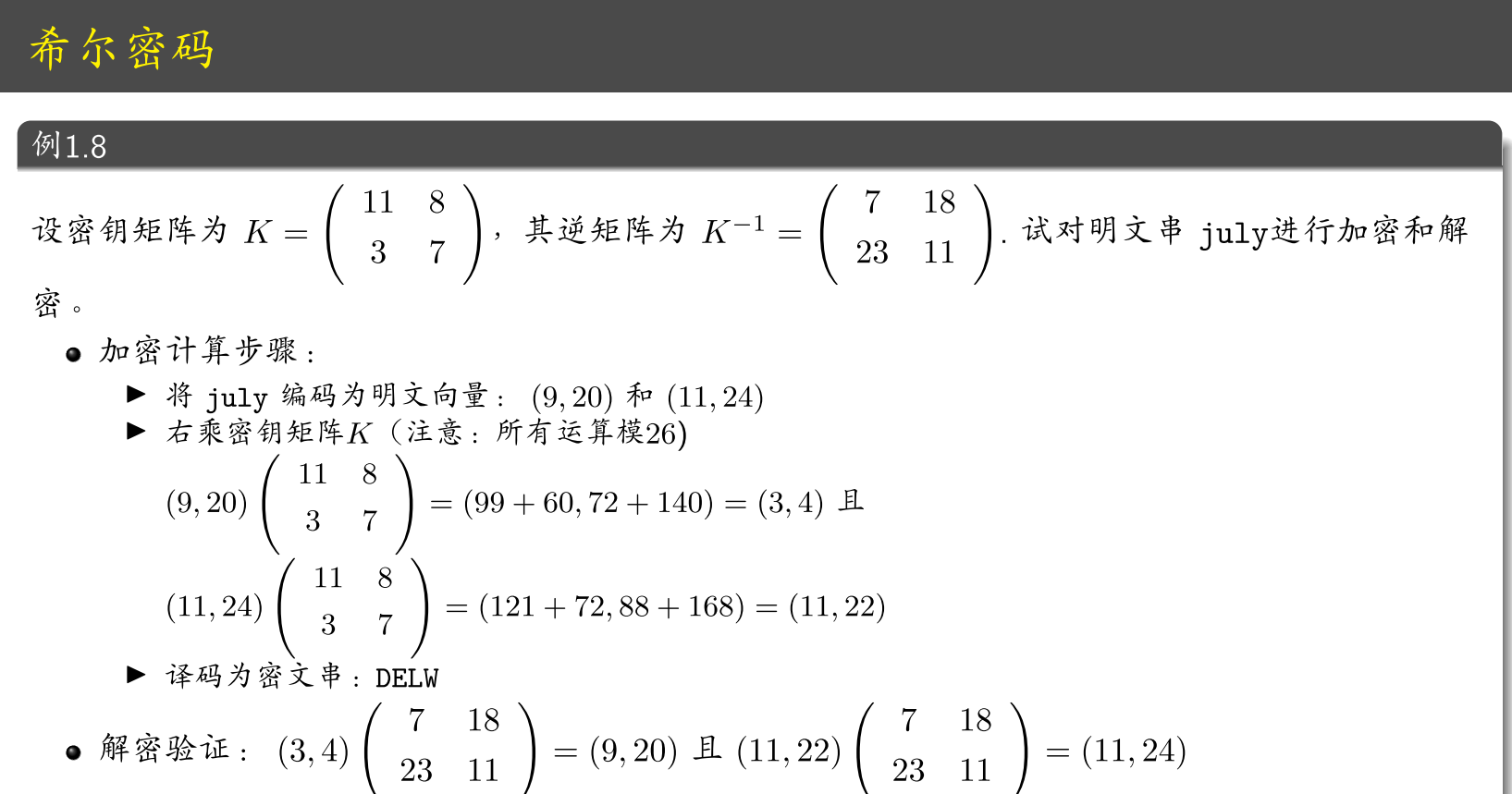## 置换密码

$\displaylines{令m为一正整数。P=C=(\mathbb{Z}_{26})^m，K是由所有定义在集合{1,2,...,m}上的置换组成。 \\ 对任意的密钥(即置换)\pi，定义 \\ e_\pi(x_1,x_2,...,x_m)=(x_{\pi(1)},x_{\pi(2)},...,x_{\pi(m)}) \\ d_\pi(x_1,x_2,...,x_m)=(x_{\pi^{-1}(1)},x_{\pi^{-1}(2)},...,x_{\pi^{-1}(m)}) \\ 其中\pi^{-1}为置换\pi的逆置换}$

# 第二章 加密方案的安全性

## 现代密码学的基本原则

• 对“安全”的定义必须是公式化的、表述严格且精确的。
• 若密码学方案的安全性依赖于某个未被证明的假设，这种假设必须精确陈述，且假设需要尽可能少。
• 密码学方案应该有严格的安全证明。

## 完善保密性

### 无条件安全（Perfect Secrecy）

• 密钥生成子算法（Gen）

• 加密子算法（Enc）

• 解密子算法（Dec）

#### 敌手不可区分性

$$PrivK^{eav}$$表示一个给定的$$\Pi$$$$\mathcal{A}$$的实验，实验定义如下：

1.敌手$$\mathcal{A}$$输出一对信息$$m_0,m_1 \in \mathcal{M}$$.

2.由$$Gen$$产生一个随机密钥$$k$$，并且从$$0,1$$里面随机选择一个比特$$b$$.然后，计算密文$$c \leftarrow Enc_k(m_b)$$交给$$\mathcal{A}$$.

3.$$\mathcal{A}$$输出一个比特$$b'$$

4.若$$b'=b$$，定义实验的输出为$$1$$；否则为$$0$$.若输出为$$1$$，记为$$Privk^{eav}_{\mathcal{A},\Pi}=1$$，此时称$$\mathcal{A}$$成功.

### 一次一密

$$Gen$$$$\mathcal{K}=\left\{0,1\right\}^l$$里面按均匀分布选取一个二进制串

$$Enc_k(m)$$：输出$$c:=k\oplus m$$

$$Dec_k(c)$$：输出$$m:=k\oplus c$$

• 密钥长度和明文长度一样长
• 密钥只能使用一次
• 密钥管理异常复杂

### 完善保密性小结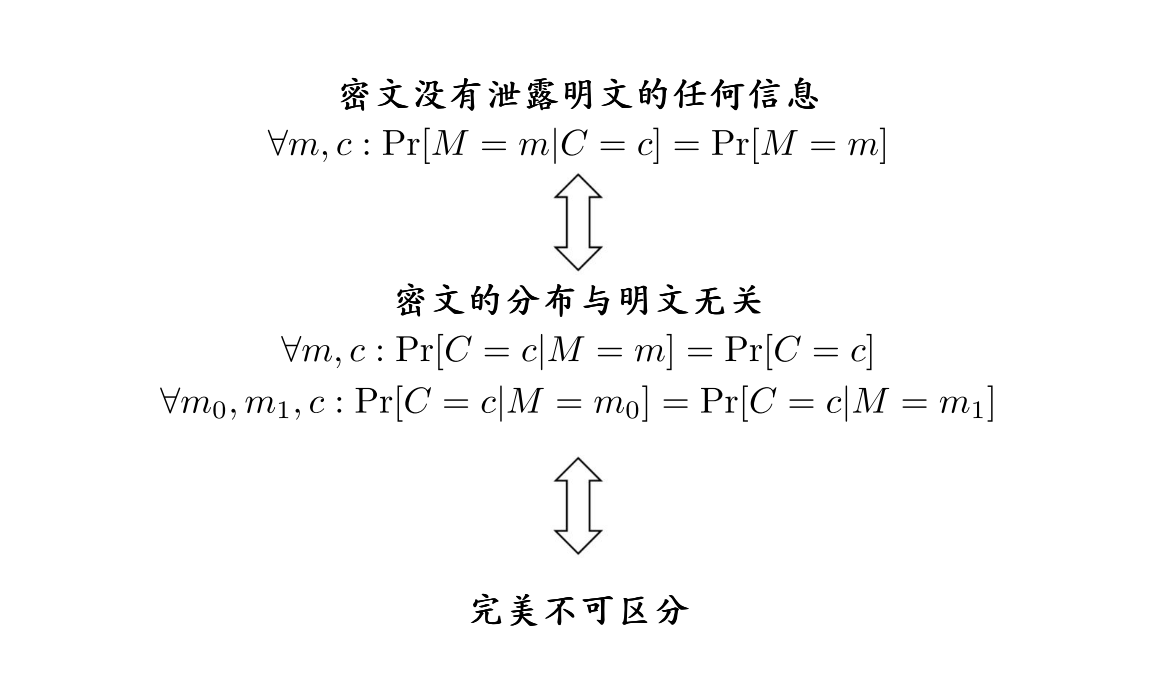## 计算安全性

### 可忽略函数

• 渐进表示：当安全参数$$n$$足够长时，PPT（概率多项式时间）敌手攻破密码算法的概率快速趋近于0
• 形式化定义：可忽略函数

### 窃听不可区分实验

1.给定输入$$1^n$$给敌手$$\mathcal{A}$$, $$\mathcal{A}$$输出一对长度相等的信息$$m_0,m_1$$. 2.运行$$Gen(1^n)$$生成一个密钥$$k$$,选择一个随机比特$$b$$,计算$$c\leftarrow Enc_k(m_b)$$并交给$$\mathcal{A}$$.这里的$$c$$称为挑战密文 3.$$\mathcal{A}$$输出一个比特$$b'$$ 4.若$$b=b'$$，则实验输出为$$1$$;否则为$$0$$.若$$Privk^{eav}_{\mathcal{A},\Pi}(n)=1$$，则称$$\mathcal{A}$$成功

$Pr[Privk^{eav}_{\mathcal{A},\Pi}(n)=1]\leq \frac{1}{2}+negl(n)$ 则称对称加密方案$$\Pi=(Gen,Enc,Dec)$$在窃听者存在的情况下不可区分的加密。

## 伪随机性

### 伪随机数发生器(PRG)

$$\mathcal{l}(·)$$为多项式，令$$G$$为确定多项式时间算法。这个算法满足：对于任何输入$$s \in \left\{0,1\right\}^n$$$$G$$输出一个长度为$$\mathcal{l}(n)$$的字符串。如果满足下面的两个条件，则称$$G$$是一个伪随机发生器：

1.扩展性：对于每个$$n$$，有$$\mathcal{l}(n)>n$$

2.伪随机性：对于所有的PPT区分器$$\mathcal{D}$$来说： $|\Pr[D(r) = 1] - \Pr [D(G(s)) =1]| \leq negl(n)$ 其中$$r$$是从$$\left\{0,1\right\}^{\mathcal{l}(n)}$$中均匀随机选择的；s是从$$\left\{0,1\right\}^n$$中均匀随机选择的。

$$\mathcal{l}(n)$$称为扩展因子

#### 用PRG构造定长加密方案

• $$Gen$$：随机选择$$k \in \left\{0,1\right\}^n$$
• $$Enc$$$$c:=G(k)\oplus m$$
• $$Dec$$$$m:=G(k)\oplus c$$

$\varepsilon(n) \triangleq \Pr[PrivK^{eav}_{\mathcal{A},\Pi}(n) = 1] - \frac12$ 显然，如果$$\mathcal{A}$$能攻破$$\Pi$$(可以判断出密文来源)，那么$$\varepsilon(n)$$是不可忽略的。

1.运行$$\mathcal{A}(1^n)$$$$\mathcal{A}$$产生一对消息$$m_0,m_1$$交回给$$\mathcal{D}$$

2.$$\mathcal{D}$$随机选择一个比特$$b$$，令$$c:=\mathcal{w} \oplus m_b$$

3.把$$c$$发给$$\mathcal{A}$$$$\mathcal{A}$$猜测$$c$$是源于$$m_0$$还是$$m_1$$，将猜测结果$$b'$$交给$$\mathcal{D}$$.如果$$b'=b$$，则$$\mathcal{D}$$输出$$1$$；否则输出$$0$$.

# 第三章 分组密码

## 分组加密的构造方法

### 分组密码

• 将明文分成等长的分组（64bit, 128bit）

• 对每个分组采用相同的加密算法进行加密

• 算法一般是交替使用混淆和扩散技术

### 实际构造方法

• 代换-置换网络（SPN，Substitution Permutation Network）

• Feistel网络

• 雪崩效应

#### 代换-置换网络（SPN）

• 代换$$\pi_S$$$$\left\{0,1\right\}^l \rightarrow \left\{0,1\right\}^l$$
• 置换$$\pi_P$$$$\left\{1,…,lm\right\} \rightarrow \left\{1,…,lm\right\}$$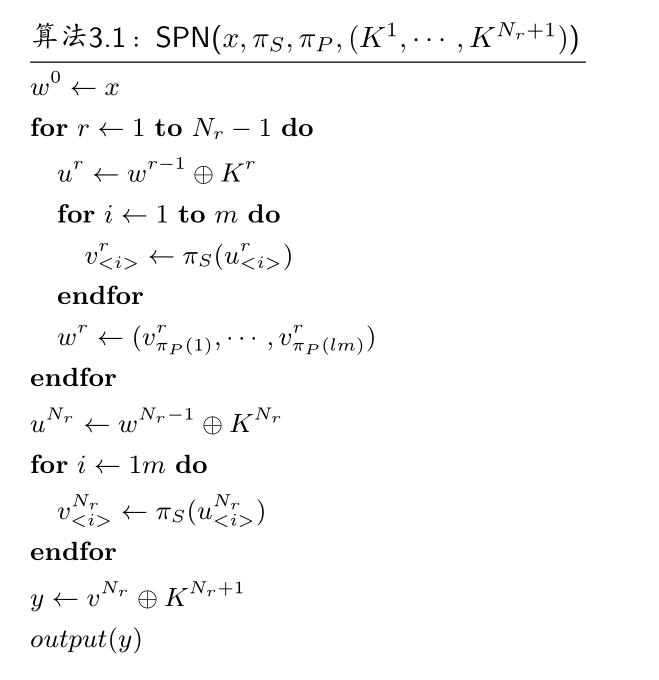• 二进制明文串$$\mathcal{w_{i-1}}$$与密钥$$K_i$$异或得到$$u_i$$
• $$u_i$$中每四位查询代换表——$$S$$盒进行代换得到$$v_i$$
• $$v_i$$每一位查询置换表——$$P$$盒进行置换得到$$w_i$$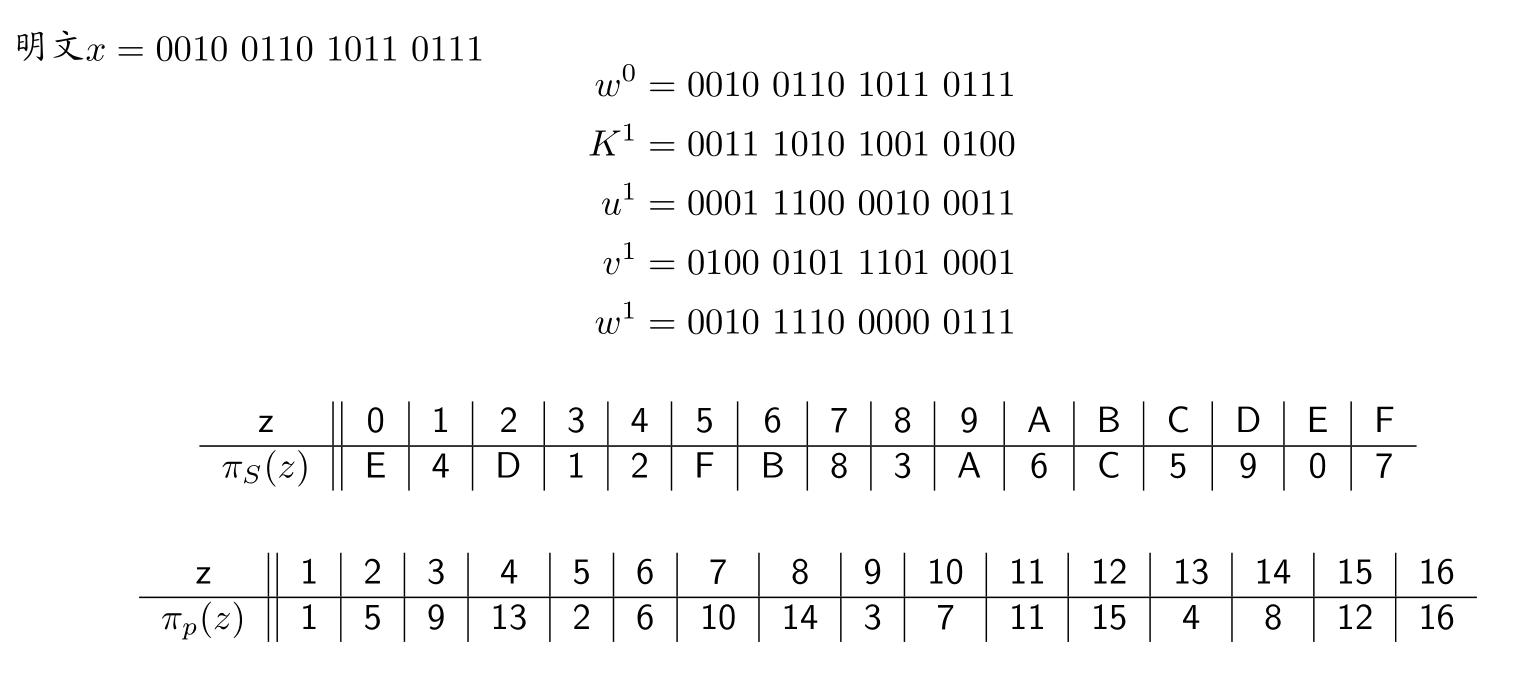#### Feistel密码结构

• 明文分组分为$$L_0$$$$R_0$$，通过$$n$$次循环处理后，再结合起来生成密文分组。
• 每第$$i$$次循环都以上一循环产生的$$L_{i-1}$$$$R_{i-1}$$$$K$$产生的子密钥$$K_i$$作为输入
• 所有循环的结构都相同，其方法是先对数据的右半部分和子密钥应用函数$$F$$，然后对函数输出结果和数据的左半部分取异或。
• 在置换之后，执行由数据两部分互换构成的交换。
• 解密过程与加密过程基本相同。规则如下：用密文作为算法的输入，但以相反顺序使用子密钥 $$K_i$$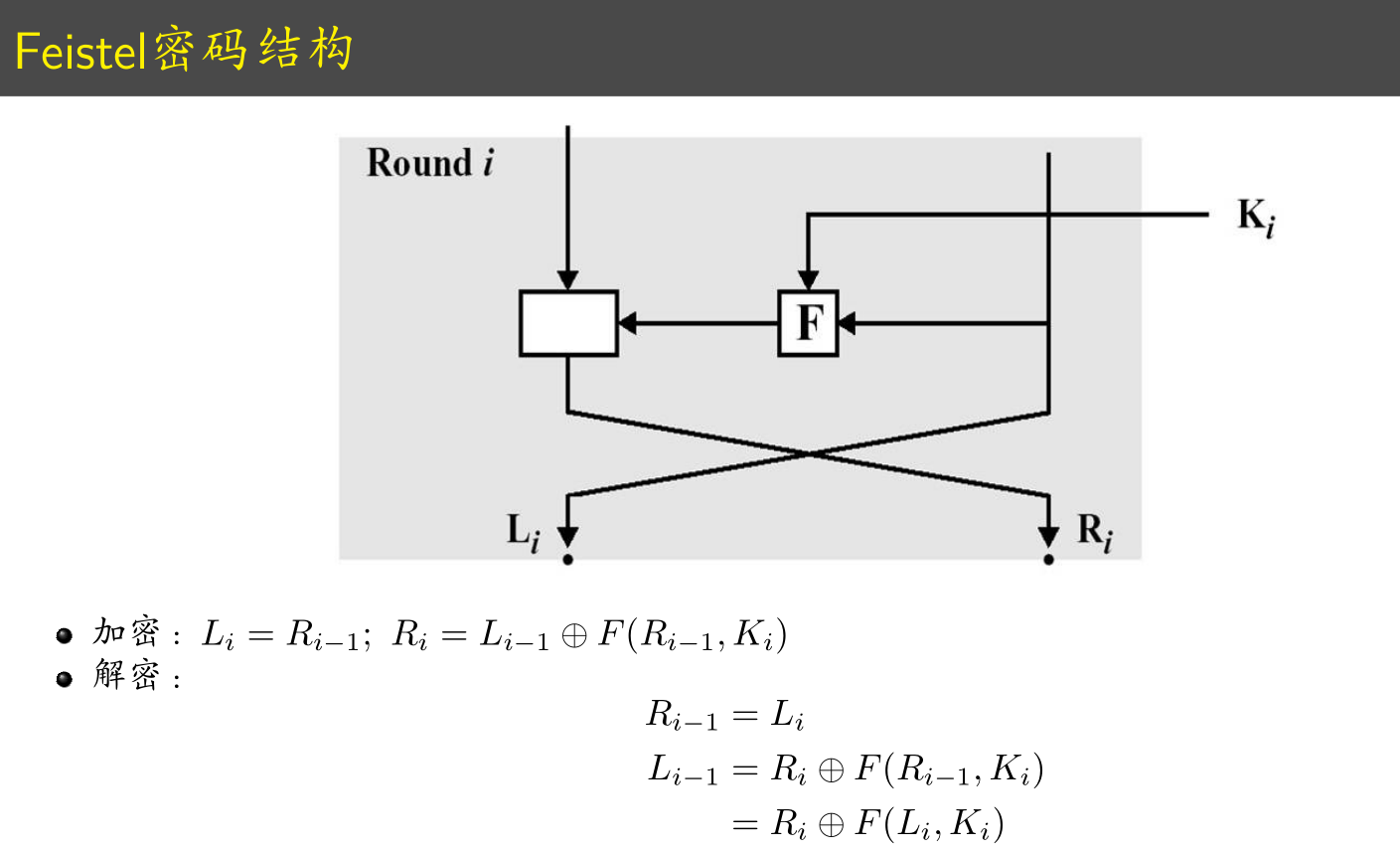### 数据加密标准（DES, Data Encryption Standard）

#### 概要

128比特分组，128、192和256位密钥。如果密钥长度是128比特，则轮数是10，如果密钥长度是192比特，则轮数是12，如果密钥长度是256比特，则轮数是14.

AES和SPN网络结构非常的相似。每一轮都是用了密钥混合、代换和置换。AES在每一轮包括一个额外的线性变换“MixColumn”

AES的操作单元以字节为单位

AES的$$S$$盒是有限域上的操作，也可以写为一个表

#### 具体过程

• 矩阵变换
• 字节变换(SubBytes)
• 行移位(ShiftRows)
• 列混淆(MixColumns)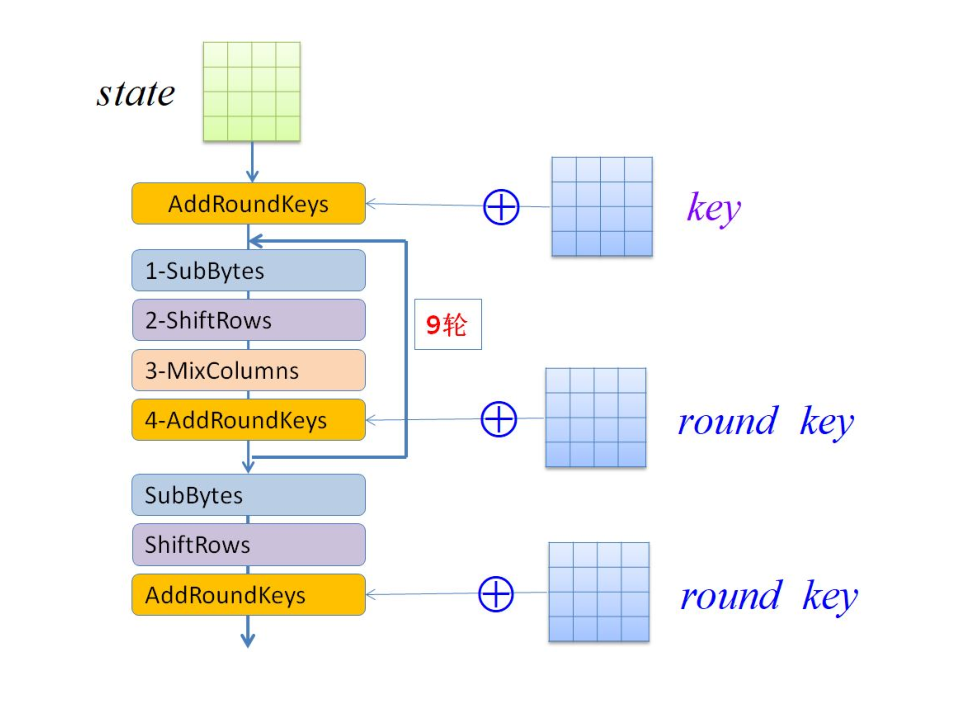##### 矩阵变换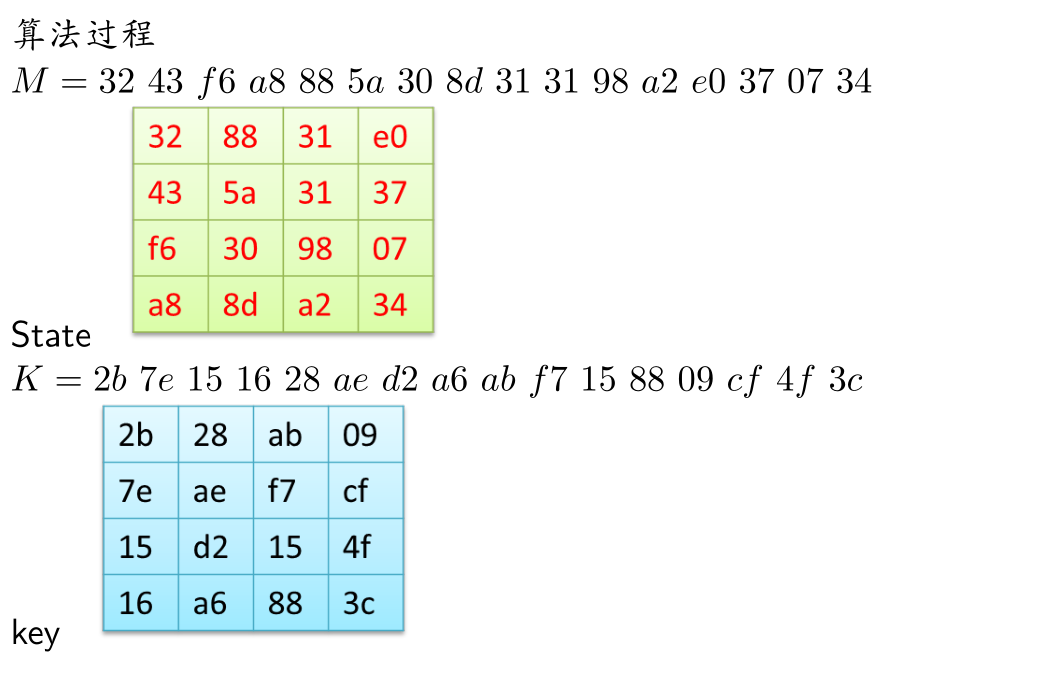##### 加轮密钥##### 字节变换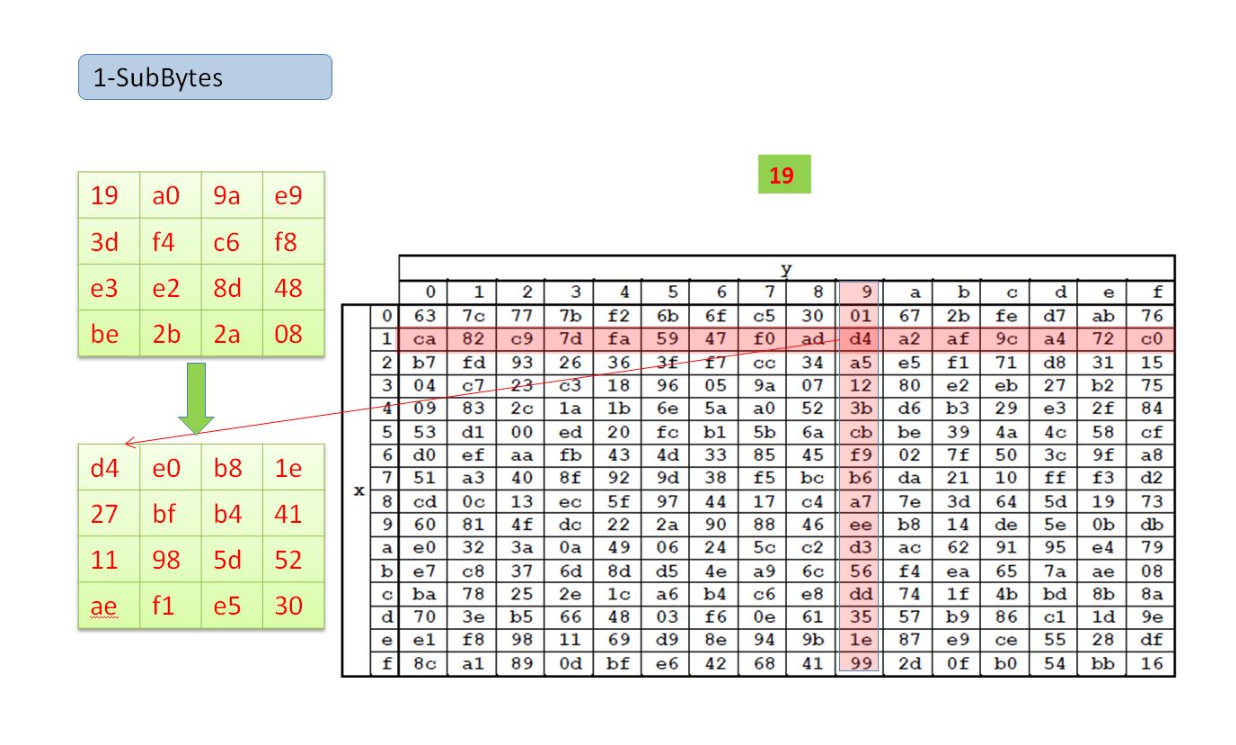19中，1对应$$S$$盒的第二行，9对应$$S$$盒的第十列，查询$$S$$盒得到变换$$19 \rightarrow d4$$

##### 行移位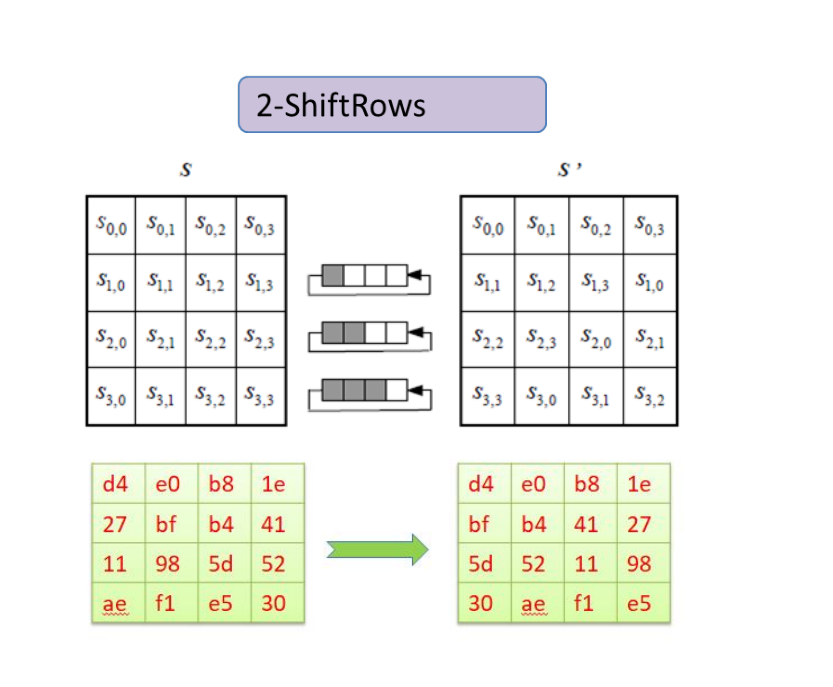##### 列混淆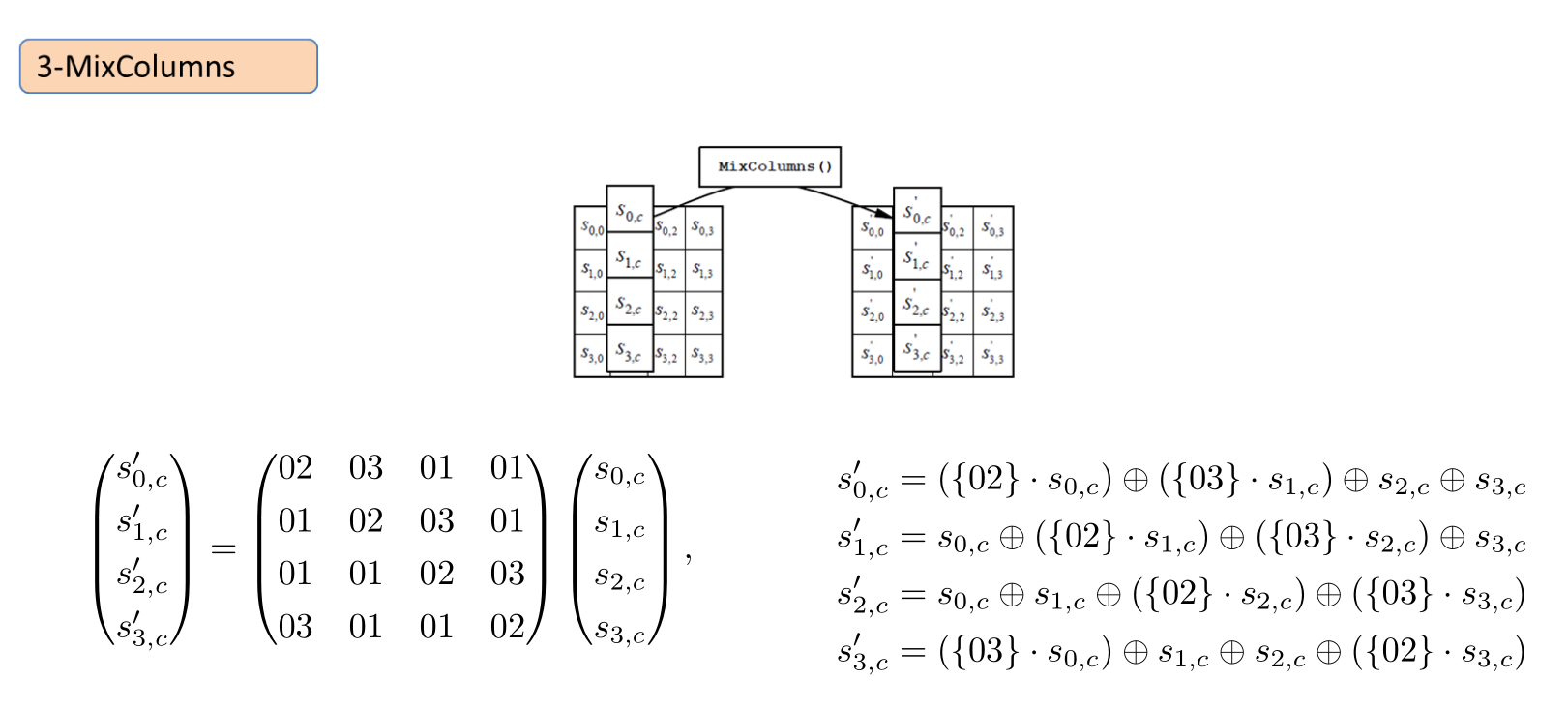##### 加轮密钥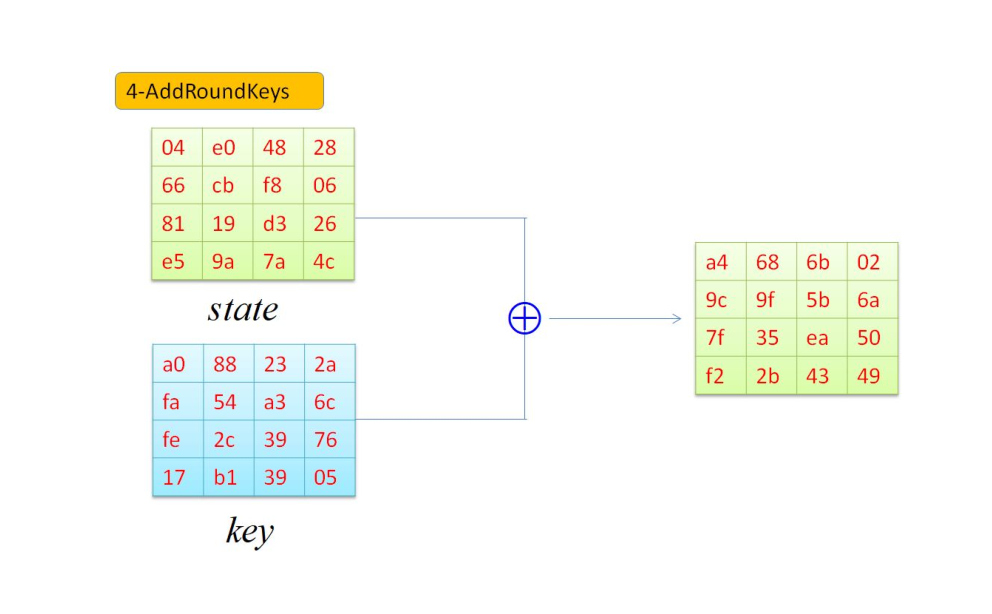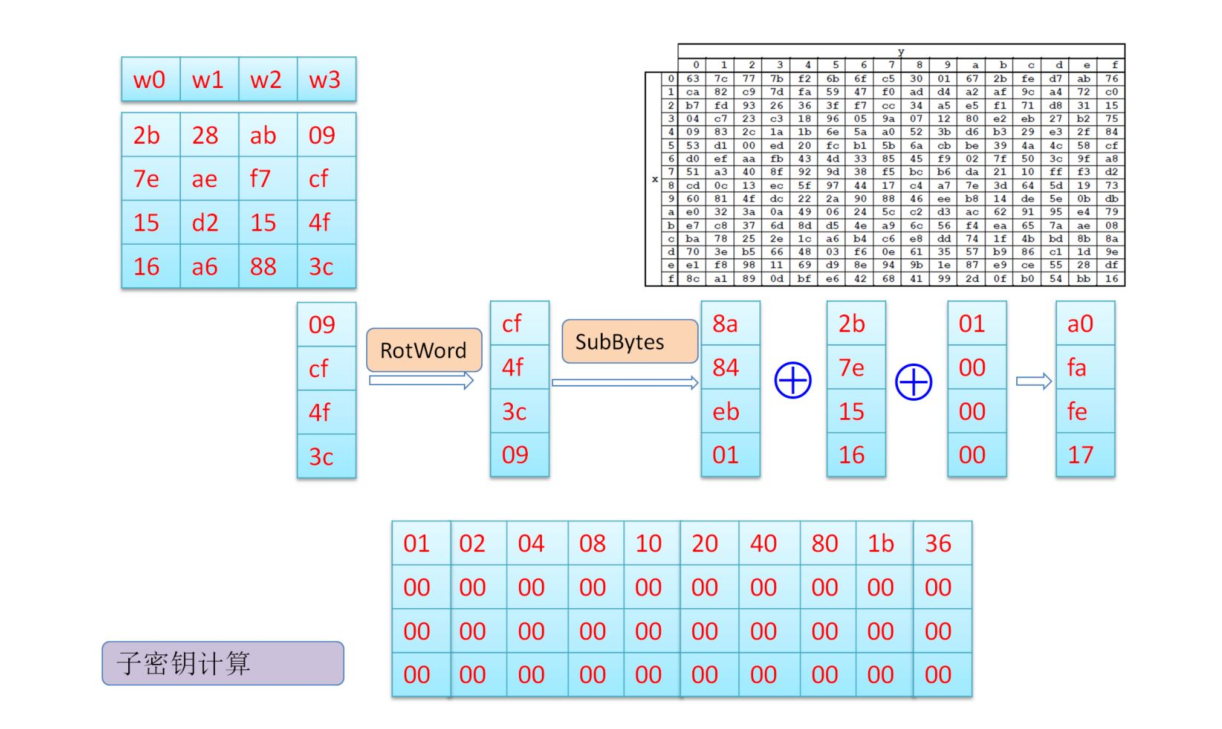## 分组加密的工作模式

### ECB模式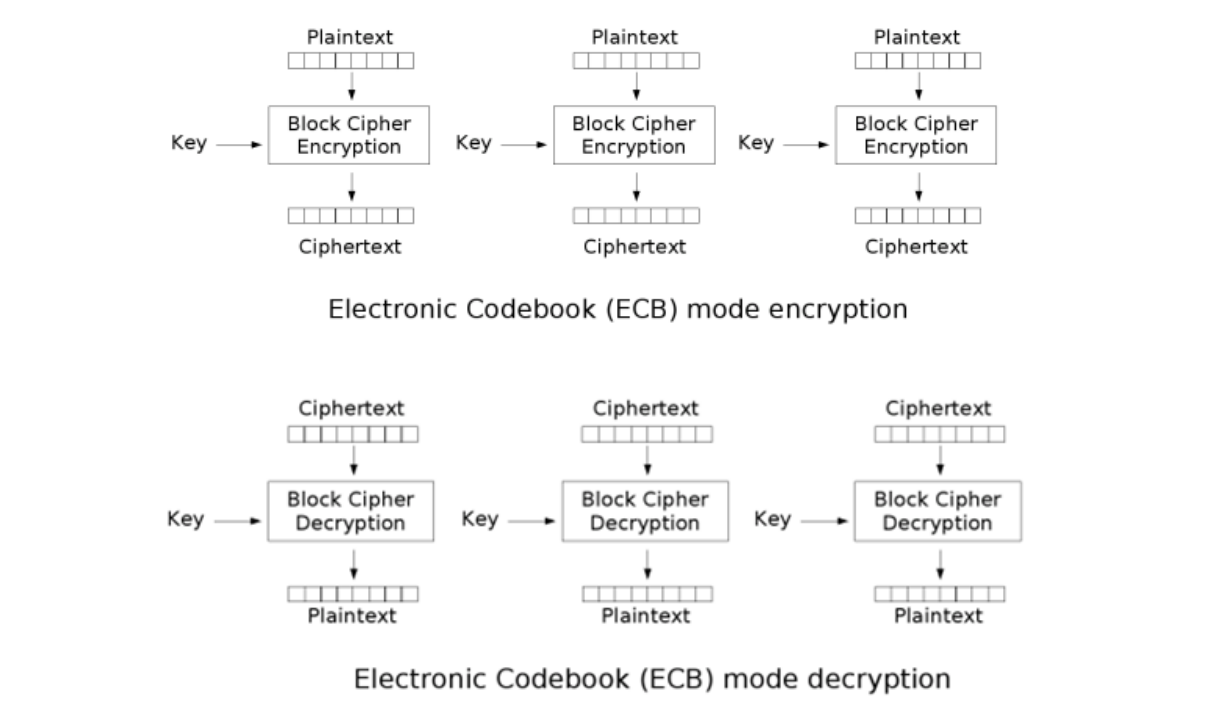• 由于相同的块会被加密成同样的密文，所以并不能很好地隐藏数据模式
• ECB模式是确定性地加密方案，所以不是CPA-安全的
• ECB模式甚至不是EAV-安全的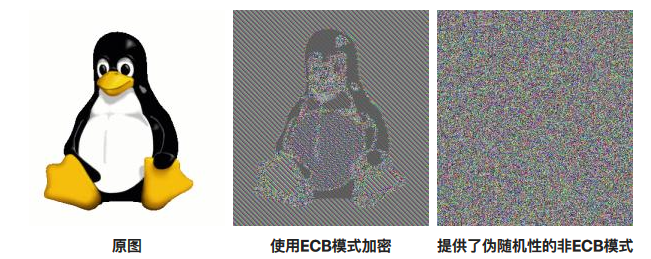### CBC模式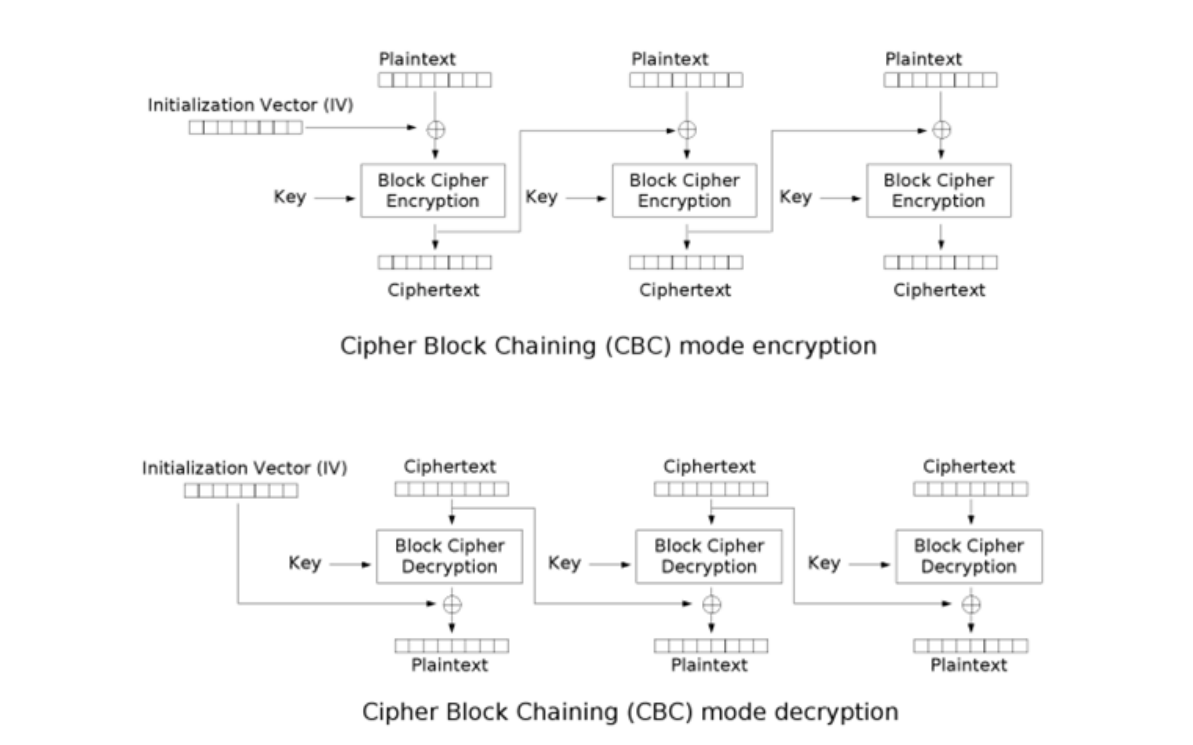$$C_i=E_k(P_i)\oplus C_{i-1}$$

$$C_0 = IV$$

$$P_i = D_K(C_i)\oplus C_{i-1}$$

$$C_0 = IV$$

• 加密过程是串行的，无法并行化
• 消息必须被填充到块大小的整数倍

### CFB模式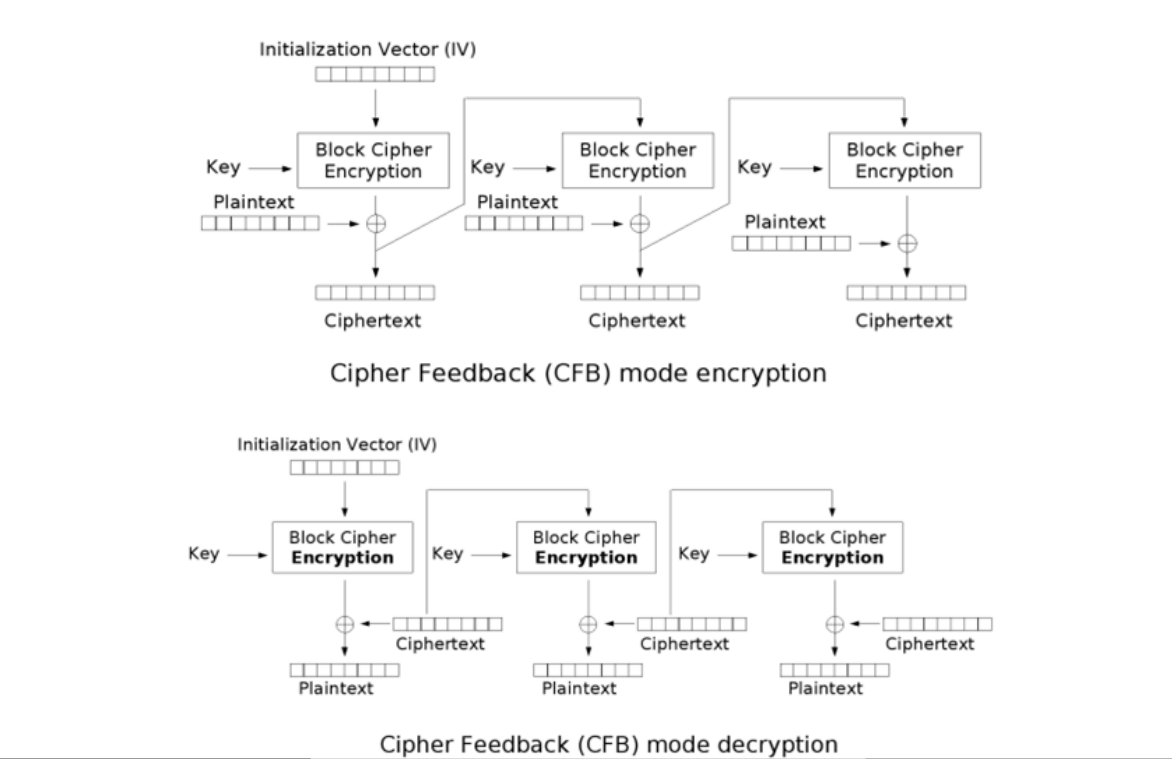$$C_i = head(E_K(S_{i-1}),x)\oplus P_i$$

$$P_i = head(E_K(S_{i-1}),x)\oplus C_i$$

$$S_i = ((S_{i-1}<<x)+C_i){\,\,}mod{\,\,}2^n$$

$$S_0 = IV$$

• 与ECB，CBC不同，CFB不需要进行填充，因为是流模式

• 与CBC类似，加密过程是串行的，因此并不能并行化，解密可以使用并行化

### OFB模式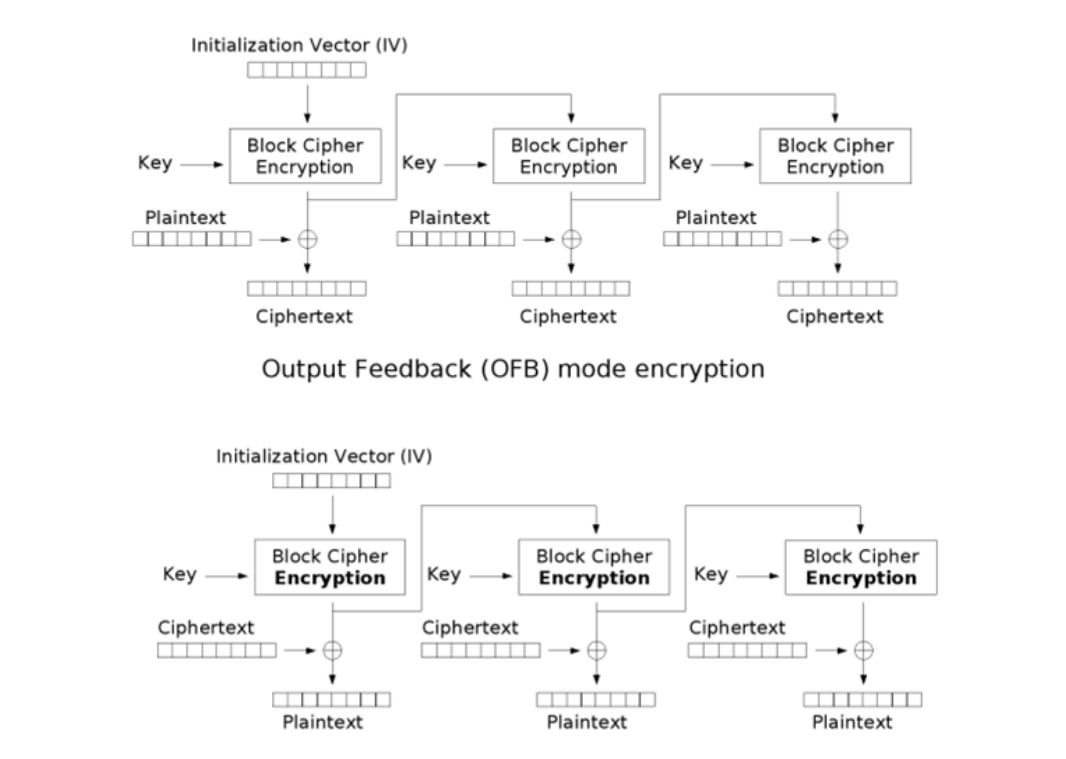$$C_i = P_i \oplus O_i$$

$$P_i = C_i \oplus O_i$$

$$O_i = E_K(O_{i-1})$$

$$O_0 = IV$$

### CTR模式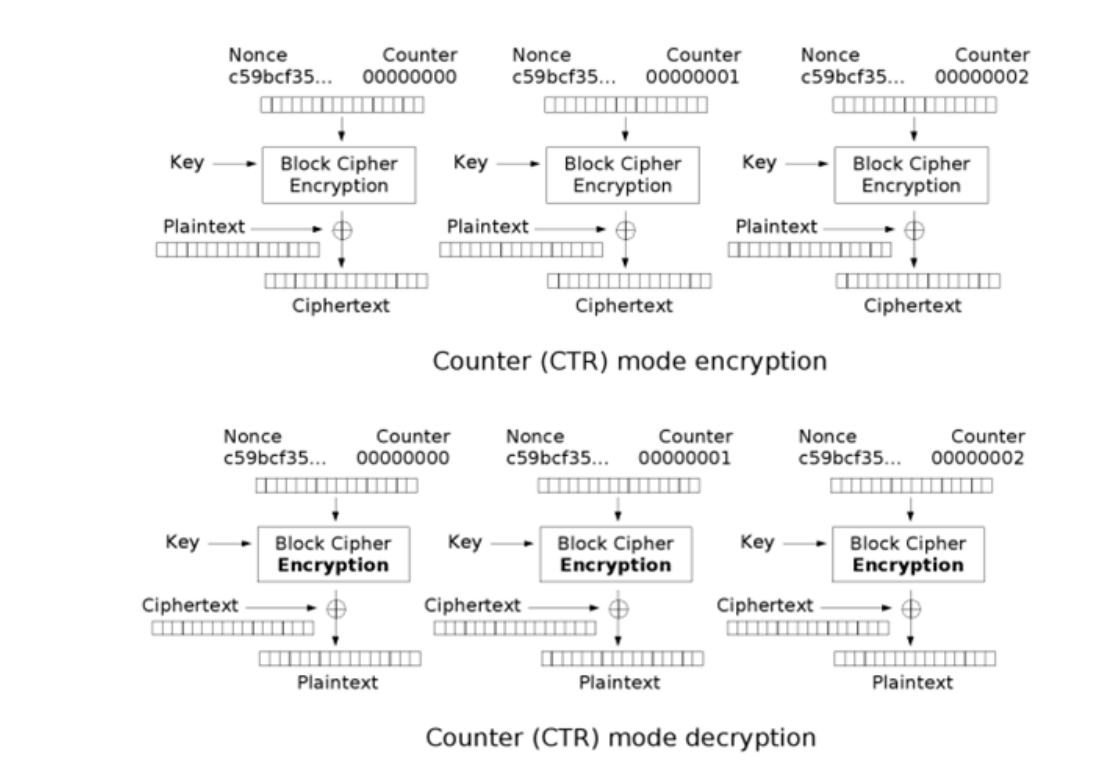# 第四章 消息认证码和散列函数

## 消息认证码（$$Mac$$）

• 密钥生成子算法$$Gen$$ —— 算法输入：安全参数$$n$$；算法输出：满足特定分布的密钥$$k$$
• 消息认证码子算法$$Mac$$ —— 算法输入：密钥$$k$$和消息$$m \in \left\{0,1\right\}^*$$；算法输出：$$Mac$$标签$$tag$$$$t \leftarrow Mac(k,m)$$
• 验证子算法$$Vrfy$$ —— 算法输入：密钥$$k$$，消息$$m$$，标签$$t$$；算法输出：$$b=Vrfy(k,m,t)$$验证通过为$$1$$，验证失败为$$0$$

### 消息认证码的安全性

1. 敌手$$\mathcal{A}$$发送消息$$m_i \in \left\{0,1\right\}^*$$

2. 消息认证算法计算$$Gen(n)$$获得密钥$$k$$$$Mac$$语言机计算标签$$tag_i = Mac(k,m_i)$$并返回给敌手$$\mathcal{A}$$，令$$Q$$表示所有敌手$$\mathcal{A}$$查询过的所有明文$$m_i$$的集合

3. 敌手$$\mathcal{A}$$伪造出$$(m^*,t^*)$$

4. $$Vrfy_k(m^*,t^*)=1$$$$m^* \notin Q$$，则敌手$$\mathcal{A}$$伪造实验成功，实验输出$$Mac-forge_{\mathcal{A},\Pi}(n)=1$$；否则输出$$Mac-forge_{\mathcal{A},\Pi}(n)=0$$

$\Pr[Mac-forge_{\mathcal{A},\Pi}(n)=1] \leq negl(n)$

### 构造具体的消息认证码方案

#### CBC-MAC

CBC-MAC是运用CBC模式对消息$$m=(m_1,m_2,…,m_n)$$进行加密，获得的$$tag_i = Mac(k, tag_{i-1} \oplus m_i)$$$$tag = tag_n$$

## 散列函数（HASH函数）

• 已知$$x$$，计算$$y=f(x)$$容易
• 已知$$y$$，计算$$x$$使得$$y = f(x)$$困难
• y是定长的

HASH函数产生的定长的输出又可称为“摘要

HASH函数的特点：

• 任意给定$$h$$，则很难找到$$M$$使得$$h=H(M)$$ ，即给出HASH值，要求输入$$M$$在计算上是不可行的，即运算过程是不可逆的，这种性质称为函数的单向性，或抗原像攻击
• 给定消息$$M$$和其HASH值$$H(M)$$，要找到另一个$$M'$$，且$$M\not={M'}$$，使得$$H(M) = H(M')$$在计算上是不可行的，这条性质被称为抗弱碰撞性，或抗第二原像攻击
• 找到两个不同的消息$$M \not ={M'}$$，使得它们的摘要值$$H(M)=H(M')$$，在计算上是不可行的，这条性质被称为抗强碰撞性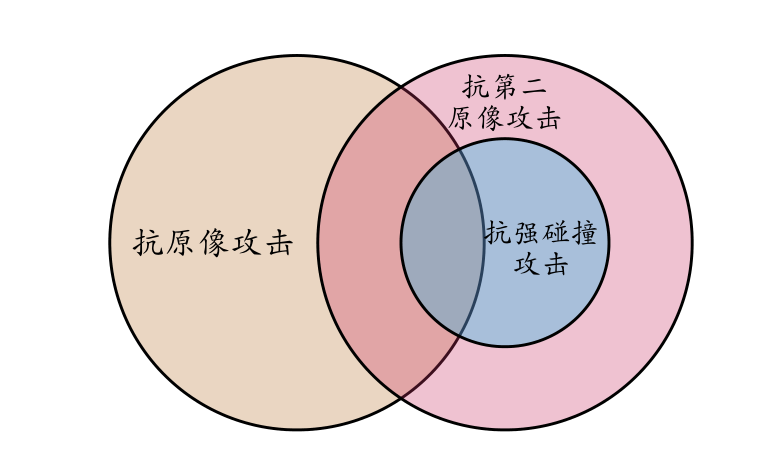### 构造HASH函数

#### Merkle-Damgård结构

Merkle-Damgård结构（MD结构）利用压缩函数进行迭代处理

MD结构流程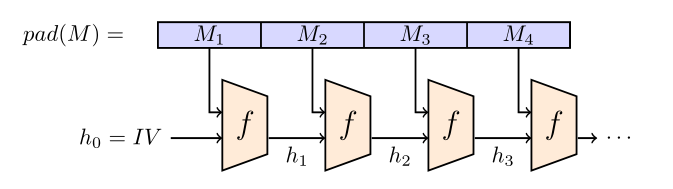MD结构对输入消息进行填充，让消息变成固定长度的整数倍（比如512或1024），通常是用0来填充。为了避免压缩函数删掉后面额外的0导致填充和不填充后的结果是一样的情况，要将第一个填充位改为1。

#### Sponge结构

Sponge结构流程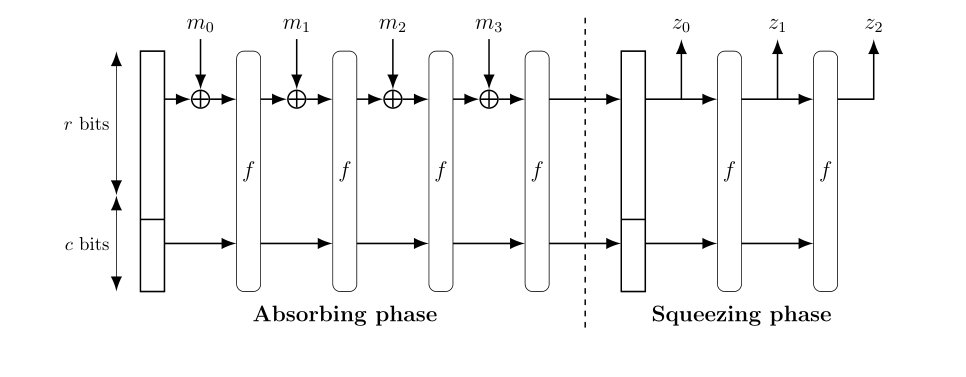### 利用HASH函数构造Mac方案

$\displaylines{Mac(k,m_1||m_2)\\ =HASH(k||m_1||m_2)\\ =f(HASH(k||m_1), m_2)\\ =f(Mac(k,m_1),m_2)\\}$

# 第五章 公钥密码学

• 一个密钥是加密密钥，发送者用来加密消息，是公开的，称为公开密钥
• 另一个密钥是解密密钥，接收者用来解密恢复明文，是秘密持有的，称为解密密钥

## Diffie-Hellman 密钥交换算法

Diffie-Hellman密钥交换算法基于离散对数的计算困难，因为离散对数的分布基本没有什么规律

$$GF(p)$$上，有一个原根$$g$$.现给定$$g,h\not={0}$$，要求找出$$x$$，使得

$g^x \equiv h \pmod p$

Diffie-Hellman密钥交换算法的思路：既然计算离散对数是困难的，那么只要把信息隐藏在离散对数中，通过传递$$g^x$$可以使得窃听者无法获得$$x$$

1.Alice和Bob通过任意渠道，达成共识$$(g,p)$$.其中$$p$$是一个质数，$$g$$是它的一个原根

2.Alice想一个随机数$$a$$，计算得到$$A=g^a$$，将$$A$$发送给Bob

3.Bob想一个随机数$$b$$，计算得到$$B=g^b$$，将$$B$$发送给Alice

4.Alice得到$$B$$后，计算$$B^a$$作为密钥

5.Bob得到$$A$$后，计算$$A^b$$作为密钥

## RSA加密方案

• 单向：已知明文$$x$$和加密密钥$$k$$很容易计算出密文$$y$$，但知道$$y$$和公钥$$k$$很难计算出明文$$x$$
• 陷门：已知密文$$y$$和解密密钥$$d$$，可以很容易计算出明文$$x$$. 解密密钥$$d$$是单向函数的陷门

RSA密码体制定义如下：

$$N=p \times q$$，其中$$p$$$$q$$为素数。设$$\mathcal{M} = \mathcal{C} = \mathbb{Z}_n$$，密钥$$K = \left\{(n,p,q,e,d):ed \equiv 1 \pmod{\phi(n)}\right\}$$，定义

• 加密：$$Enc_K(x) = x^e \pmod n$$
• 解密：$$Dec_K(y) = y^d \pmod n$$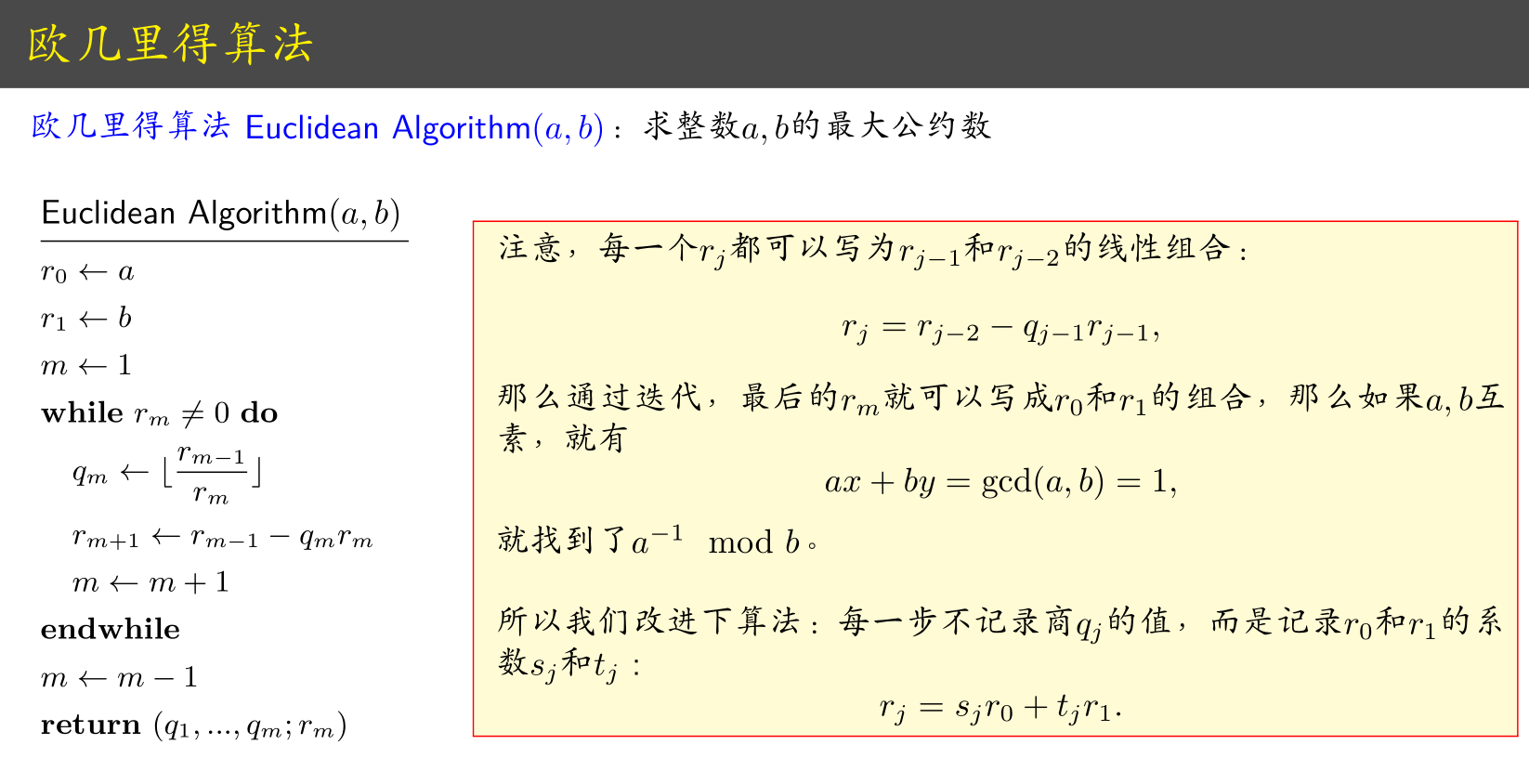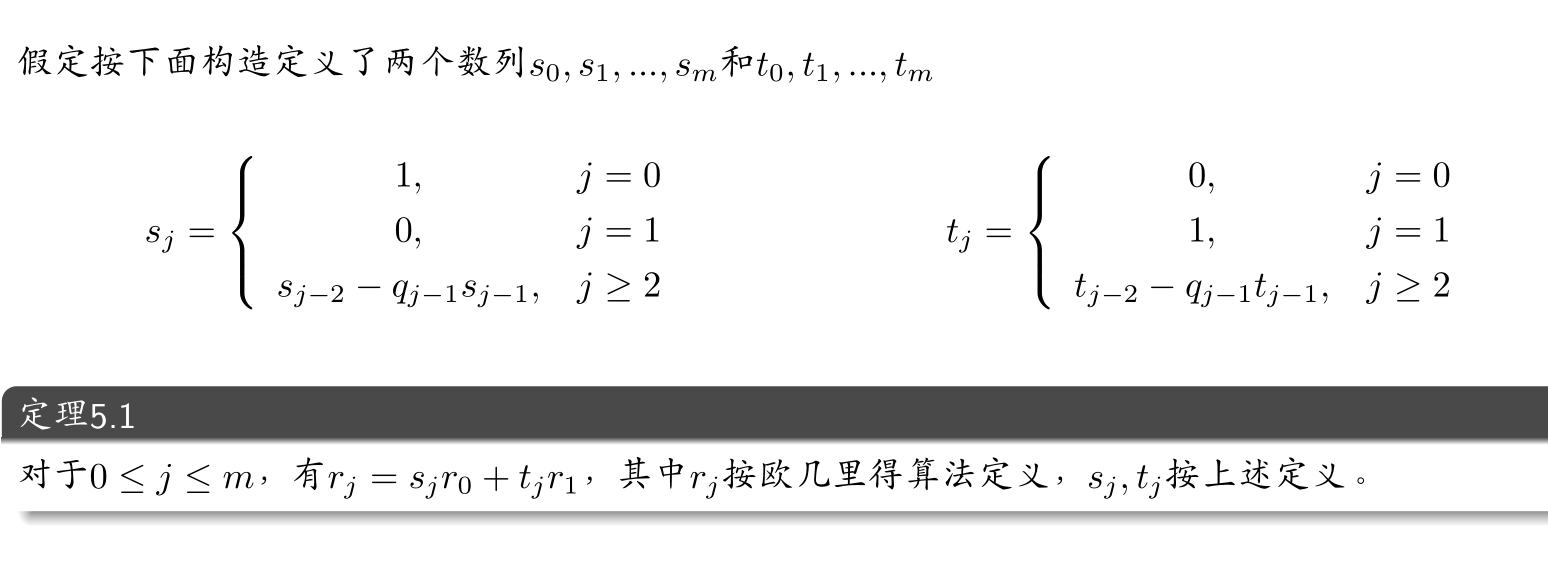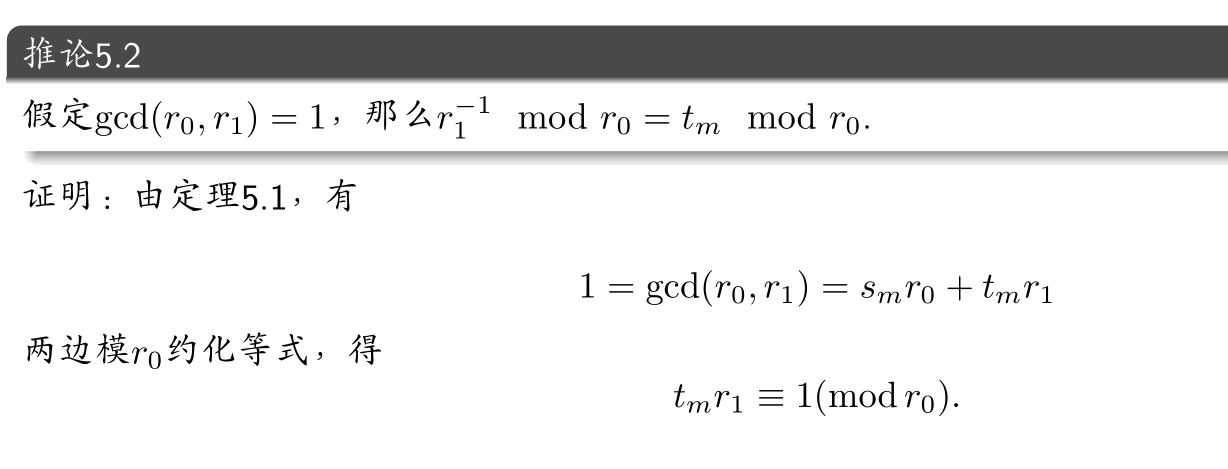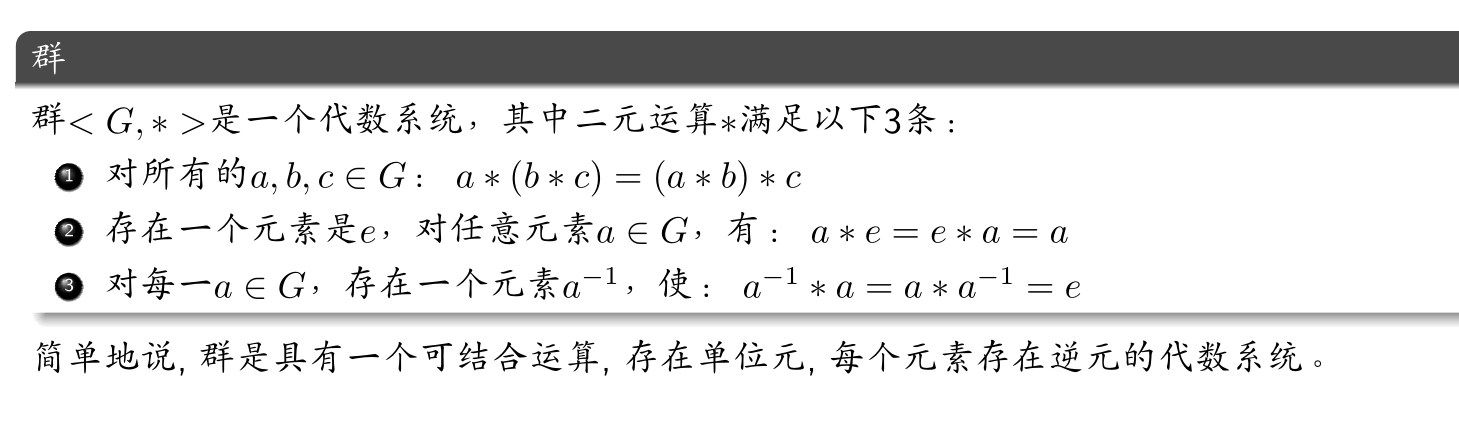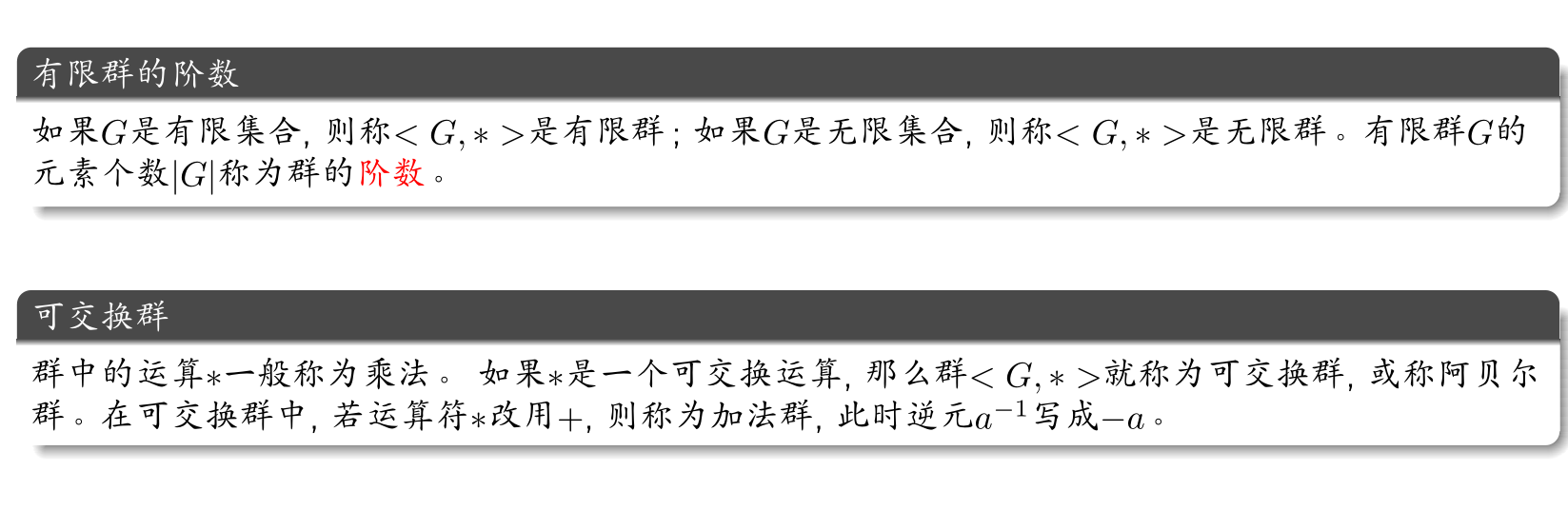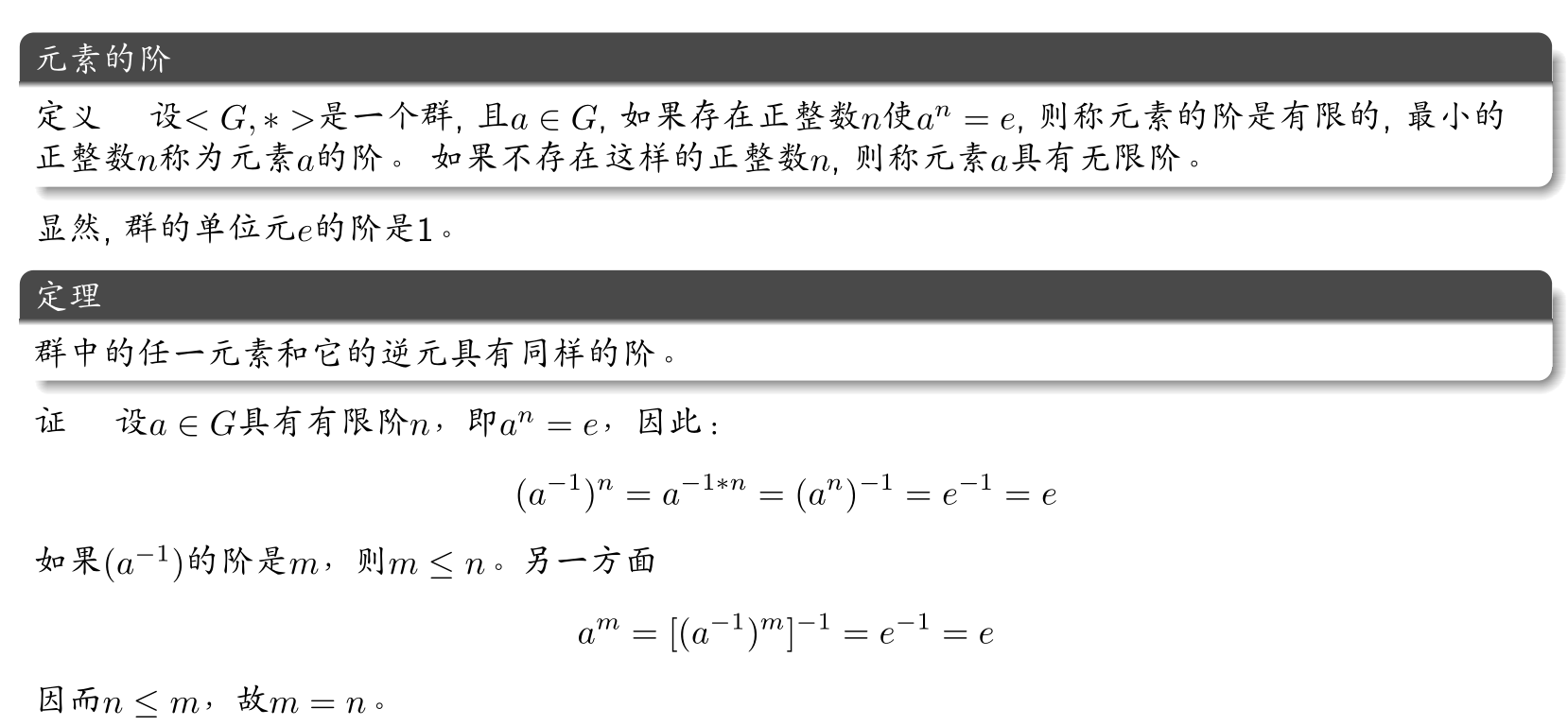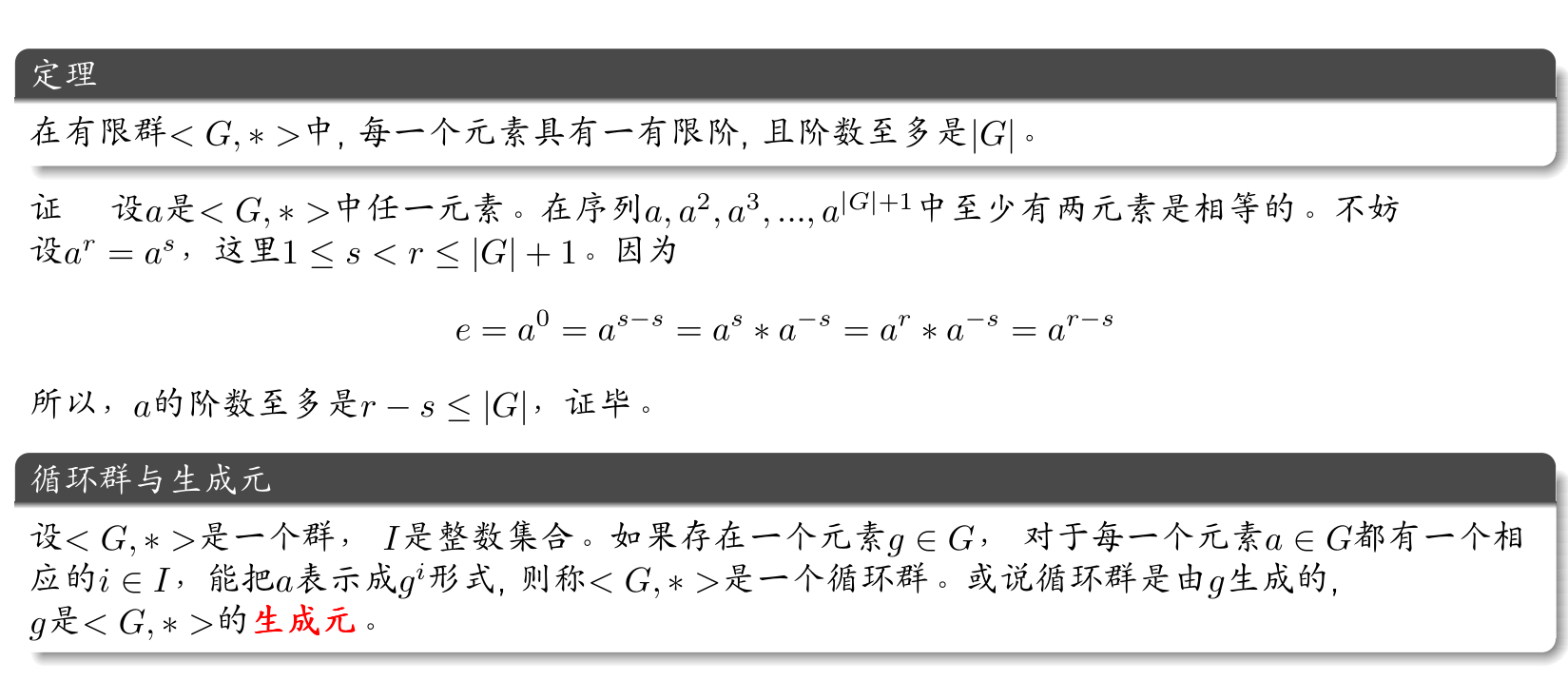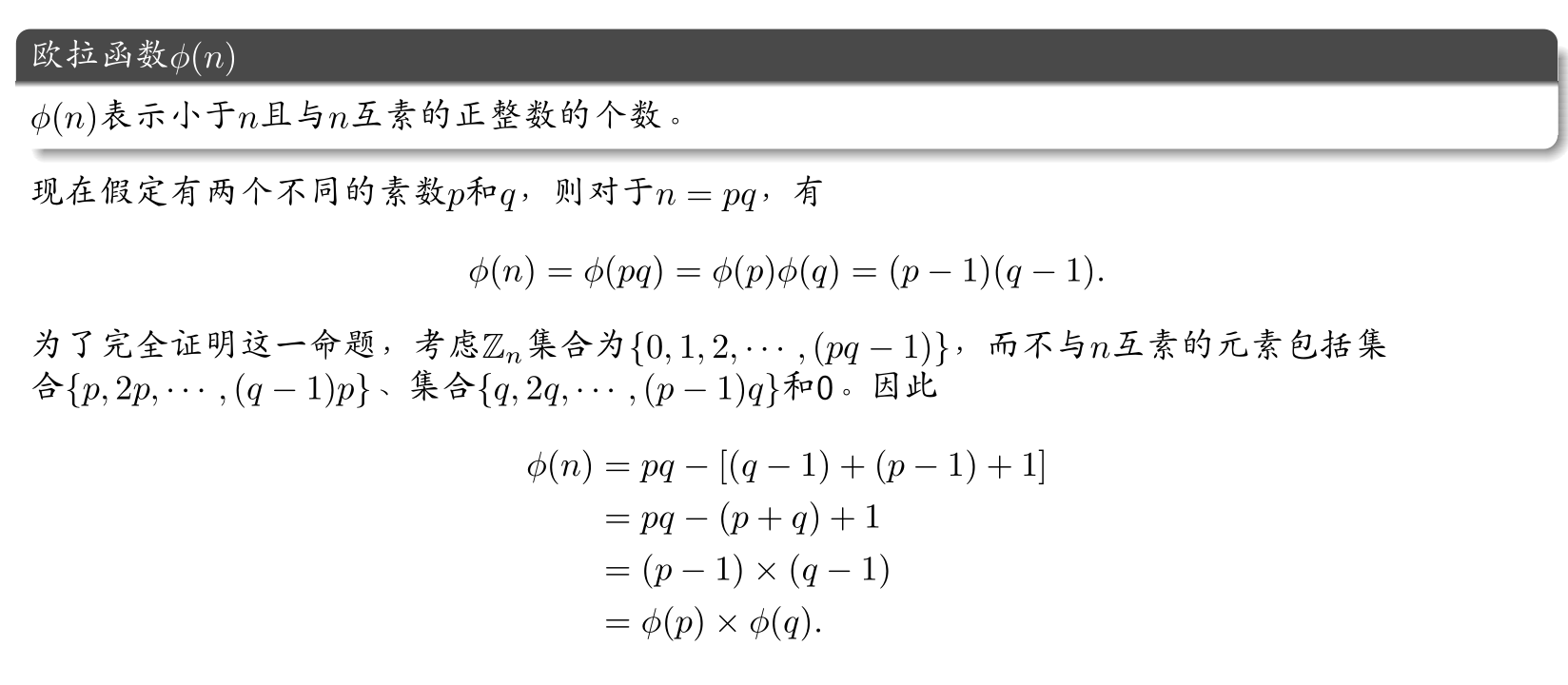RSA算法具体过程：

$$n \leftarrow pq$$，且$$\phi(n) \leftarrow (p-1)(q-1)$$

$$d \leftarrow e^{-1} \mod \phi(n)$$

### OAEP vs. OAEP+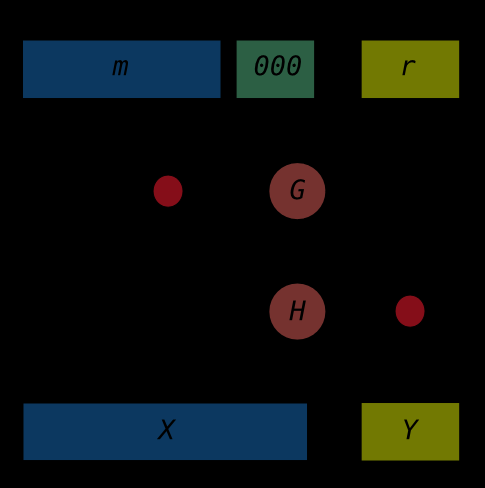1.用$$k_1$$位长的$$0$$串将消息$$m$$填充至$$n-k_0$$位的长度

2.随机生成$$k_0$$位长的串$$r$$

3.用$$G$$$$k_0$$位长的$$r$$扩展至$$n-k_0$$位长

4.$$X = (m||00...00) \oplus G(r)$$

5.用$$H$$$$n-k_0$$位长的$$X$$缩短至$$k_0$$位长

6.$$Y = r \oplus H(X)$$

7.输出$$f(X||Y)$$

## 素性检测

### 初等数论基础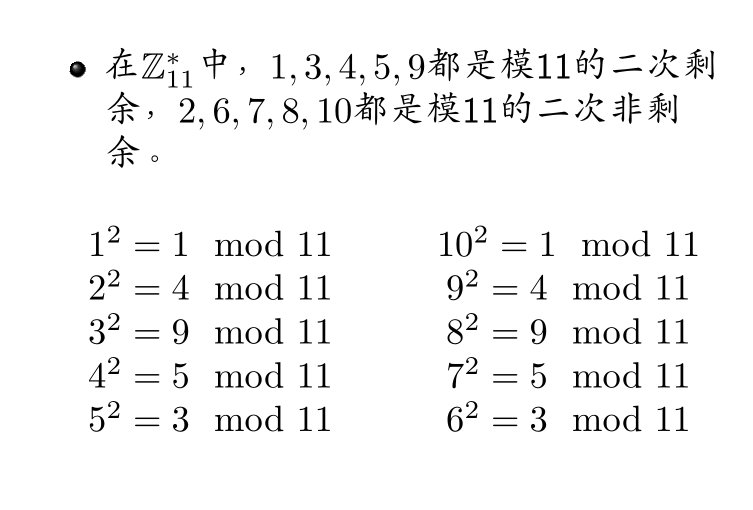Legendre符号

\left(\frac{a}{p}\right) = \left\{ \begin{aligned} 1, & {\,\,\,\,\,\,\,\,}p \nmid a {\,\,} 且a是模p的二次剩余 & \\ -1,& {\,\,\,\,\,\,\,\,}p \nmid a {\,\,} 且a不是模p的二次剩余 & \\ 0, & {\,\,\,\,\,\,\,\,}p \mid a \end{aligned} \right.

Euler准则：

$$p$$为一个奇素数，$$a$$为一个正整数 a^{(p-1)/2} \equiv \left(\frac{a}{p}\right) \equiv \left\{ \begin{aligned} 1 \pmod p, & {\,\,\,\,\,\,\,\,} 若x^2 \equiv a \pmod p 有解 \\ -1\pmod p, & {\,\,\,\,\,\,\,\,} 若x^2 \equiv a \pmod p 无解 \end{aligned} \right.

$$p$$为素数，$$a$$为任意自然数，则

$a^p \equiv a \pmod p$

$$gcd(a,p) = 1$$，则$$p | (a^{p-1} - 1)$$，即

$a^{p-1} \equiv 1 \pmod p$

$$p$$为素数，那么下面的同余方程仅有两个解，$$a=1$$$$a = p - 1$$ $a^2 \equiv 1 \pmod p$

### Miller-Rabin素性检测

$$x$$为奇数，则$$x-1$$为偶数，可以表示为$$2^sd$$，其中$$d$$为奇数

$a^{2^sd} \equiv 1 \pmod x$

Miller-Rabin素性检测基于上面的逆否，如果能找到这样一个$$a$$不满足上原理，那么$$x$$就不是素数，这样的$$a$$称为$$x$$是合数的一个凭证，否则$$a$$可能是证明$$x$$是素数的“强伪证”。

# 第五章 离散对数问题和ElGamal密码体制

## ElGamal密码体制

Elgamal密码体制基于Diffie-Hellman密钥交换算法。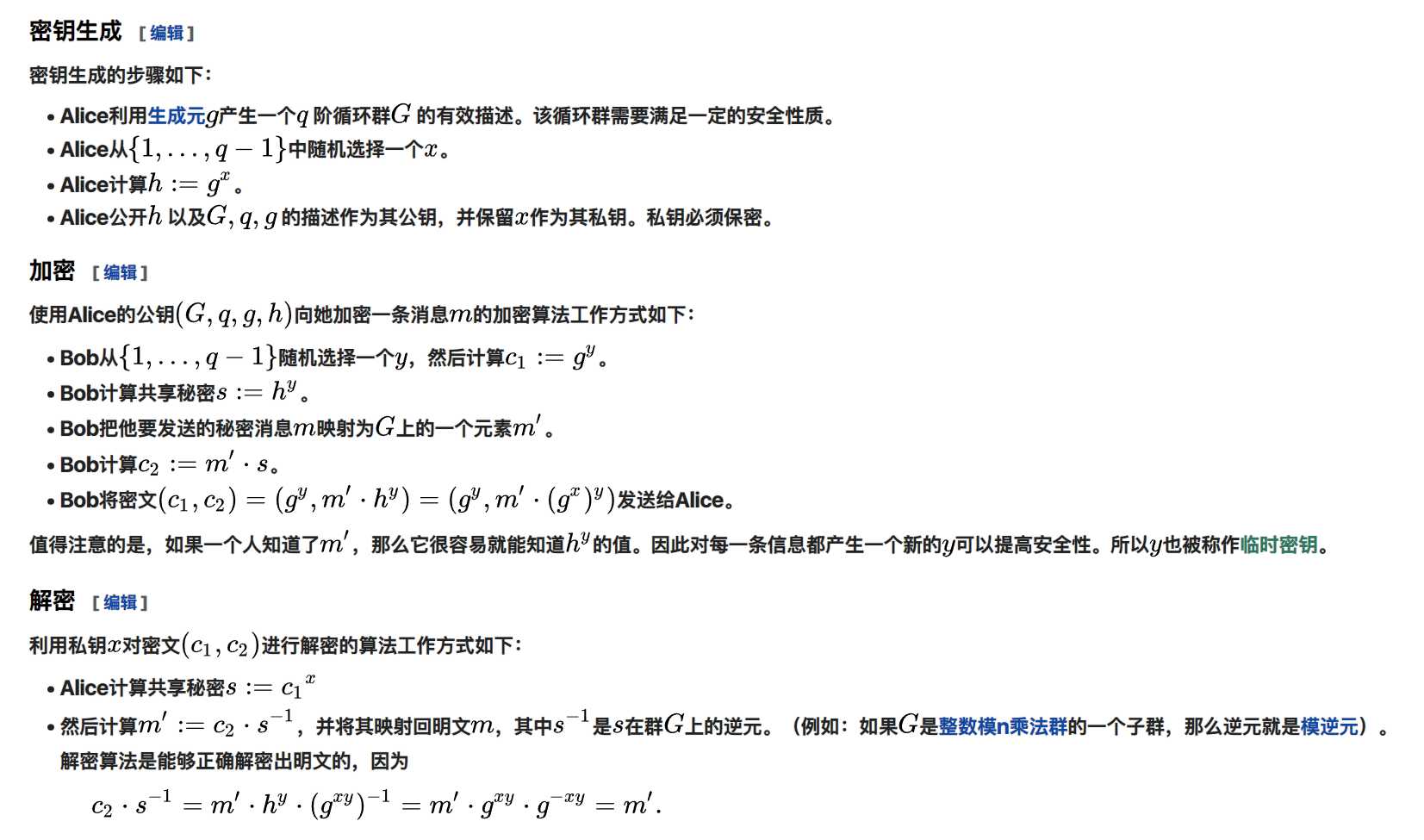## 椭圆曲线加密

### 定义

#### 初始定义

$\left\{(x,y)|x,y \in y^2=x^3+ax+b \right\}$

#### 椭圆曲线上的加法定义

$$P+Q=R'$$

#### 无穷远点

$P+Q=\mathcal{O}$

#### 模素数意义下的椭圆曲线定义

$$p>3$$且为素数，在$$\mathbb{Z}_p$$上的同余方程

$y^2 \equiv x^3 + ax + b \pmod p$

$4a^3+27b^2 \not={0}$

### 运算

$$\mathbb{Z}_p$$有限域上的椭圆曲线加法运算满足下列性质

$nP = P + P + P + \dots + P$

\begin{aligned} x_3 & = \lambda^2 - x_1 - x_2\\ y_3 & = \lambda(x_1 - x_3) - y_1 \end{aligned}

\lambda = \left\{ \begin{aligned} & (y_2-y_1)(x_2-x_1)^{-1} & {\,\,\,\,\,\,\,\,} P \not={Q} \\ & (3x^2_1+a)(2y_1)^{-1} & {\,\,\,\,\,\,\,\,} P=Q \end{aligned} \right.

### 椭圆曲线的离散对数问题

$$E$$是有限域$$\mathbb{Z}_p$$上的椭圆曲线，$$P,Q \in E(\mathbb{Z}_p)$$. 椭圆曲线离散对数问题是指：找到一个整数$$n$$使得$$Q=nP$$.我们记

$n = log_P(Q)$

$E:Y^2 = X^3 + 8X + 7$

# 第六章 数字签名

## 数字签名安全模型

$\rm{Pr}[\rm{Sig} - \rm{forge}_{\cal{A},\Pi}(n)=1] \leq \rm{negl}(n)$

## 常见数字签名算法

### RSA签名方案

$$\rm{KeyGen}$$. $$\cal{K}=\left\{(n,p,q,a,b):n=pq,p和q是素数,ab \equiv 1 \pmod{\phi(n)},公钥(n,b),私钥(p,q,a)\right\}$$

$$\rm{Sig}$$. 对于消息$$m$$，用私钥计算$$m$$的签名 $y = {\rm{Sig}}_{\cal{K}}(x)=x^a \mod n$

$$\rm{Vrfy}$$. 给定消息$$m$$和签名$$\sigma$$，用公钥验证 ${\rm Vrfy_{\cal{K}}}(x,y)=1 \iff x = y^b \mod n$

### ElGamal签名方案

$$\rm KeyGen$$. 设$$\alpha \in {\Bbb{Z}_p}$$是一个生成元，$${\cal{P}}={\Bbb{Z}^*_{p}} \times {\Bbb{Z}^*_{p-1}}$$，定义 ${\cal{K}} = \left\{(p,\alpha,b,\beta):\beta = \alpha ^ b, 公钥是(p,\alpha, \beta), 私钥是b \right\}$

$$\rm Sig$$. 对于消息$$x$$，选择一个秘密随机数$$r \in {\Bbb Z}^*_{p-1}$$，计算 $\gamma = \alpha ^ r \mod p, {\,\,\,\,}\delta = (x-b\gamma)r^{-1} \mod{(p-1)}$

$$\rm Vrfy$$. 对于给定签名$$(\gamma, \delta)$$和消息$$x$$ ${\rm Vrfy}_{\cal K}(x,(\gamma,\delta))=1 \iff \beta ^ \gamma \gamma ^ \delta = \alpha ^ x \mod p$

ElGamal签名方案是非确定性的，也就是说对任意给定的的消息有很多有效的数字签名

\begin{aligned} &\beta ^ \gamma \gamma ^ \delta \equiv \alpha ^ {b \gamma} \alpha ^ {r \delta} \equiv \alpha ^ x \mod p \\ &\Rightarrow b = (x - r\delta)\gamma^{-1} \mod{(p-1)} \end{aligned}

### 数字签名算法(DSA)

$${\rm KeyGen}$$. 设$$p$$是长为$$L$$bit的素数，在$${\Bbb Z}^*_p$$上其离散对数问题是难解的，其中$$L \equiv 0 \pmod{64}$$$$512 \leq L \leq 1024$$$$q$$是能被$$p-1$$整除的$$160$$bit的素数，设$$\alpha \in {\Bbb Z}^*_p$$$$1$$$$p$$$$q$$次根。定义

${\cal K} = \left\{(p,q,\alpha,b,\beta): \beta \equiv \alpha ^ b \pmod p \right\}$

$${\rm Sig.}$$ 对于消息$$x$$$${\cal K} = \left\{(p,q,\alpha,b,\beta): \beta \equiv \alpha ^ b \pmod p \right\}$$和一个秘密随机数$$r \in {\Bbb Z}^*_p$$$$1 \leq r \leq q - 1$$，计算

\begin{aligned} &\gamma = (\alpha ^ r \mod{p}) \mod{q}\\ &\delta = ({\rm SHA-1}(x)+b\gamma)r^{-1} \mod{q} \end{aligned}

${\rm Sig}_{\cal K}(x,r) = (\gamma, \delta)$

$${\rm Vrfy.}$$$$x \in \left\{0,1\right\}^*,\gamma,\delta \in {\Bbb Z_q}$$，验证通过下面计算完成

\begin{aligned} e_1 & = {\rm SHA-1}(x)\delta^{-1} \mod{q}\\ e_2 & = \gamma \delta^{-1} \mod{q}\\ {\rm Vrfy}_{\cal K}(x,(\gamma, \delta)) = 1 & \iff (\alpha ^ {e_1} \beta ^ {e_2} \mod{p}) \mod{q} = \gamma \end{aligned}

### Schnorr签名方案

$${\rm KeyGen.}$$$$p$$是使得$${\Bbb Z}^*_p$$上离散对数问题难题的一个素数，$$q$$是能被$$p-1$$整除的素数。设$$\alpha \in {\Bbb Z}^*_p$$$$1$$$$p$$$$q$$次根，$$h:\left\{0,1\right\}^* \rightarrow {\Bbb Z}_q$$是一个安全的$${\rm Hash}$$函数，定义

${\cal K} = \left\{(p,q,\alpha,b,\beta):\beta \equiv \alpha ^ b \pmod{p}\right\}$

$${\rm Sig.}$$ 对于消息$$x$$$${\cal K} = \left\{(p,q,\alpha,b,\beta):\beta \equiv \alpha ^ b \pmod{p}\right\}$$和一个秘密随机数$$r \in {\Bbb Z}^*_p$$$$1 \leq r \leq q - 1$$，计算

\begin{aligned} &\gamma = h(x||\alpha ^ r \mod{p})\\ &\delta = r + b\gamma \mod{p} \end{aligned}

${\rm Sig}_{\cal K}(x,r)=(\gamma, \delta)$

$${\rm Vrfy.}$$$$x \in \left\{0,1\right\}^*,\gamma,\delta \in {\Bbb Z_q}$$，验证通过下面计算完成：

${\rm Vefy}_{\cal K}(x,(\gamma, \delta)) = 1 \iff h(x||\alpha^\delta\beta^{-\gamma} \mod{p}) = \gamma$

### 椭圆曲线数字签名算法(ECDSA)

$${\rm KeyGen.}$$$$p$$是一个大素数，$$E$$是定义在$${\Bbb F}_p$$上的椭圆曲线。设$$A$$$$E$$上阶为$$q$$$$q$$为素数）的一个点，使得$$<A>$$上的离散对数问题是个困难问题. 定义：

${\cal K} = \left\{(p,q,E,A,m,B):B \equiv mA, m \in {\Bbb Z}_q\right\}$

$${\rm Sig.}$$ 对于消息$$x$$$${\cal K} = \left\{(p,q,E,A,m,B):B \equiv mA, m \in {\Bbb Z}_q\right\}$$和一个秘密随机数$$k \in {\Bbb Z}^*_q$$$$1 \leq k \leq q - 1$$，计算

\begin{aligned} & kA = (u,v)\\ & r = u \mod{q}\\ & s = ({\rm SHA-1}(x)+mr)k^{-1} \mod{q} \end{aligned}

${\rm Sig}_{\cal K}(x,k)=(r,s)$ （如果$$r=0$$或者$$s=0$$，应该为$$k$$另选一个随机数）

$${\rm Vrfy.}$$$$x \in \left\{0,1\right\}^*r,s \in {\Bbb Z}^*_q$$，验证过程如下

\begin{aligned} w &= s^{-1} \mod{q}\\ i &= w{\rm SHA-1}(x) \mod{q}\\ j &= wr \mod{q}\\ (u,v) &= iA + jB\\ {\rm Vrfy}_{\cal K}(x,(r,s)) & = 1 \iff u \mod{q} = r \end{aligned}